# WO2012111160A1 - Torque distribution device, torque distribution method, torque distribution value generation method, and program - Google Patents

Torque distribution device, torque distribution method, torque distribution value generation method, and program Download PDF

## Info

Publication number
WO2012111160A1
WO2012111160A1 PCT/JP2011/053565 JP2011053565W WO2012111160A1 WO 2012111160 A1 WO2012111160 A1 WO 2012111160A1 JP 2011053565 W JP2011053565 W JP 2011053565W WO 2012111160 A1 WO2012111160 A1 WO 2012111160A1
Authority
WO
WIPO (PCT)
Prior art keywords
torque
efficiency
value
distribution
motor
Prior art date
Application number
PCT/JP2011/053565
Other languages
French (fr)
Japanese (ja)
Inventor

Original Assignee
パイオニア株式会社
Priority date (The priority date is an assumption and is not a legal conclusion. Google has not performed a legal analysis and makes no representation as to the accuracy of the date listed.)
Filing date
Publication date
Application filed by パイオニア株式会社 filed Critical パイオニア株式会社
Priority to PCT/JP2011/053565 priority Critical patent/WO2012111160A1/en
Publication of WO2012111160A1 publication Critical patent/WO2012111160A1/en

• 238000004364 calculation methods Methods 0.000 claims abstract description 34
• 230000000875 corresponding Effects 0.000 claims description 12
• 230000001276 controlling effects Effects 0.000 claims description 5
• 238000010586 diagrams Methods 0.000 description 70
• 230000001133 acceleration Effects 0.000 description 14
• 238000000034 methods Methods 0.000 description 12
• 230000037408 Distribution ratio Effects 0.000 description 10
• 238000004891 communication Methods 0.000 description 7
• 230000005484 gravity Effects 0.000 description 6
• 239000000446 fuels Substances 0.000 description 4
• 230000003247 decreasing Effects 0.000 description 3
• 238000005516 engineering processes Methods 0.000 description 3
• 241001125929 Trisopterus luscus Species 0.000 description 2
• 239000011572 manganese Substances 0.000 description 2
• 238000005457 optimization Methods 0.000 description 2
• 238000010248 power generation Methods 0.000 description 2
• 239000010426 asphalt Substances 0.000 description 1
• 230000005540 biological transmission Effects 0.000 description 1
• 230000037396 body weight Effects 0.000 description 1
• 239000000470 constituents Substances 0.000 description 1
• 229910052802 copper Inorganic materials 0.000 description 1
• 239000010949 copper Substances 0.000 description 1
• 238000006073 displacement reactions Methods 0.000 description 1
• 239000011514 iron Substances 0.000 description 1
• 229910052742 iron Inorganic materials 0.000 description 1
• 229910001416 lithium ion Inorganic materials 0.000 description 1
• 229910000652 nickel hydride Inorganic materials 0.000 description 1
• 230000008929 regeneration Effects 0.000 description 1
• 230000002040 relaxant effect Effects 0.000 description 1
• 230000000576 supplementary Effects 0.000 description 1
• 230000001052 transient Effects 0.000 description 1

## Images

•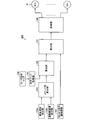•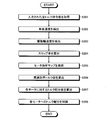•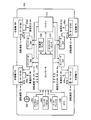•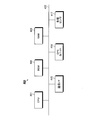•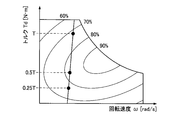•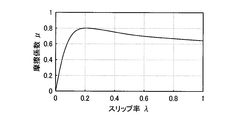•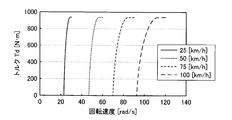•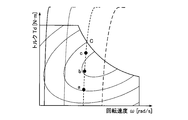•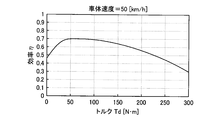•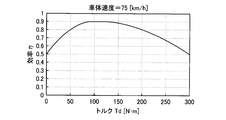•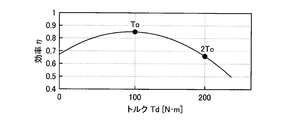•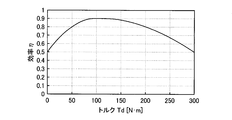•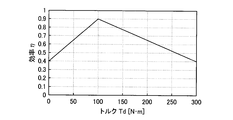••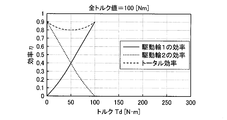•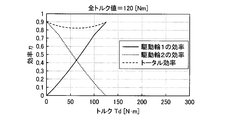•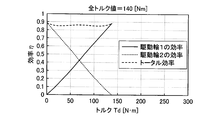•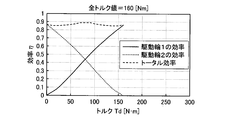•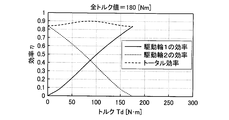•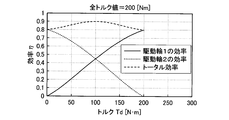•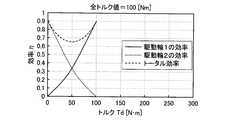•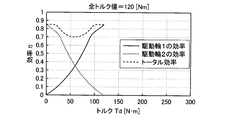•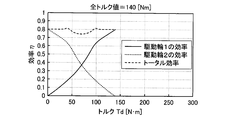•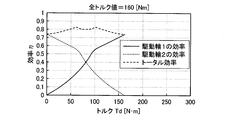•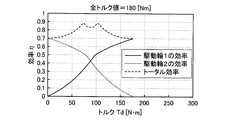•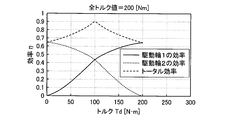•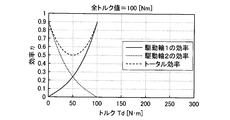•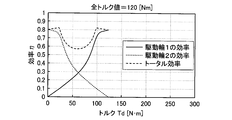•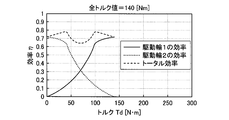•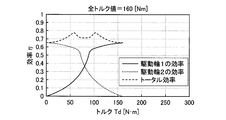•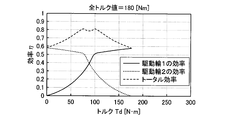•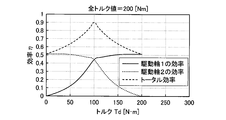•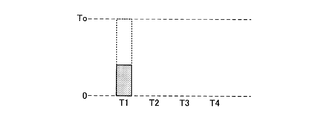•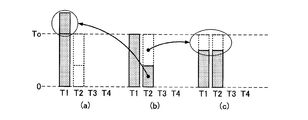•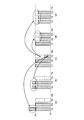•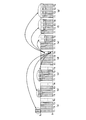•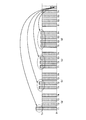•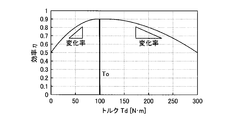•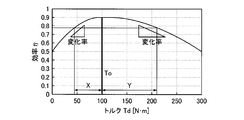•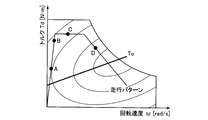•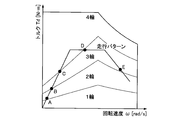•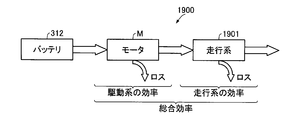•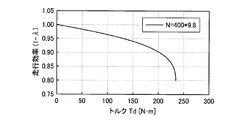•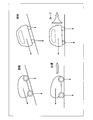•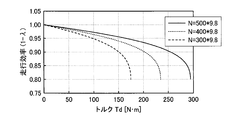•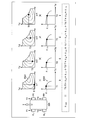•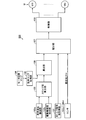•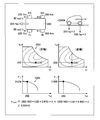•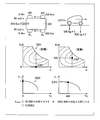## Classifications

• BPERFORMING OPERATIONS; TRANSPORTING
• B60VEHICLES IN GENERAL
• B60LPROPULSION OF ELECTRICALLY-PROPELLED VEHICLES; SUPPLYING ELECTRIC POWER FOR AUXILIARY EQUIPMENT OF ELECTRICALLY-PROPELLED VEHICLES; ELECTRODYNAMIC BRAKE SYSTEMS FOR VEHICLES IN GENERAL; MAGNETIC SUSPENSION OR LEVITATION FOR VEHICLES; MONITORING OPERATING VARIABLES OF ELECTRICALLY-PROPELLED VEHICLES; ELECTRIC SAFETY DEVICES FOR ELECTRICALLY-PROPELLED VEHICLES
• B60L15/00Methods, circuits, or devices for controlling the traction-motor speed of electrically-propelled vehicles
• B60L15/20Methods, circuits, or devices for controlling the traction-motor speed of electrically-propelled vehicles for control of the vehicle or its driving motor to achieve a desired performance, e.g. speed, torque, programmed variation of speed
• B60L15/2045Methods, circuits, or devices for controlling the traction-motor speed of electrically-propelled vehicles for control of the vehicle or its driving motor to achieve a desired performance, e.g. speed, torque, programmed variation of speed for optimising the use of energy
• BPERFORMING OPERATIONS; TRANSPORTING
• B60VEHICLES IN GENERAL
• B60KARRANGEMENT OR MOUNTING OF PROPULSION UNITS OR OF TRANSMISSIONS IN VEHICLES; ARRANGEMENT OR MOUNTING OF PLURAL DIVERSE PRIME-MOVERS IN VEHICLES; AUXILIARY DRIVES FOR VEHICLES; INSTRUMENTATION OR DASHBOARDS FOR VEHICLES; ARRANGEMENTS IN CONNECTION WITH COOLING, AIR INTAKE, GAS EXHAUST OR FUEL SUPPLY OF PROPULSION UNITS IN VEHICLES
• B60K23/00Arrangement or mounting of control devices for vehicle transmissions, or parts thereof, not otherwise provided for
• B60K23/08Arrangement or mounting of control devices for vehicle transmissions, or parts thereof, not otherwise provided for for changing number of driven wheels, for switching from driving one axle to driving two or more axles
• B60K23/0808Arrangement or mounting of control devices for vehicle transmissions, or parts thereof, not otherwise provided for for changing number of driven wheels, for switching from driving one axle to driving two or more axles for varying torque distribution between driven axles, e.g. by transfer clutch
• BPERFORMING OPERATIONS; TRANSPORTING
• B60VEHICLES IN GENERAL
• B60LPROPULSION OF ELECTRICALLY-PROPELLED VEHICLES; SUPPLYING ELECTRIC POWER FOR AUXILIARY EQUIPMENT OF ELECTRICALLY-PROPELLED VEHICLES; ELECTRODYNAMIC BRAKE SYSTEMS FOR VEHICLES IN GENERAL; MAGNETIC SUSPENSION OR LEVITATION FOR VEHICLES; MONITORING OPERATING VARIABLES OF ELECTRICALLY-PROPELLED VEHICLES; ELECTRIC SAFETY DEVICES FOR ELECTRICALLY-PROPELLED VEHICLES
• B60L15/00Methods, circuits, or devices for controlling the traction-motor speed of electrically-propelled vehicles
• B60L15/20Methods, circuits, or devices for controlling the traction-motor speed of electrically-propelled vehicles for control of the vehicle or its driving motor to achieve a desired performance, e.g. speed, torque, programmed variation of speed
• BPERFORMING OPERATIONS; TRANSPORTING
• B60VEHICLES IN GENERAL
• B60LPROPULSION OF ELECTRICALLY-PROPELLED VEHICLES; SUPPLYING ELECTRIC POWER FOR AUXILIARY EQUIPMENT OF ELECTRICALLY-PROPELLED VEHICLES; ELECTRODYNAMIC BRAKE SYSTEMS FOR VEHICLES IN GENERAL; MAGNETIC SUSPENSION OR LEVITATION FOR VEHICLES; MONITORING OPERATING VARIABLES OF ELECTRICALLY-PROPELLED VEHICLES; ELECTRIC SAFETY DEVICES FOR ELECTRICALLY-PROPELLED VEHICLES
• B60L3/00Electric devices on electrically-propelled vehicles for safety purposes; Monitoring operating variables, e.g. speed, deceleration or energy consumption
• B60L3/10Indicating wheel slip ; Correction of wheel slip
• B60L3/106Indicating wheel slip ; Correction of wheel slip for maintaining or recovering the adhesion of the drive wheels
• B60L3/108Indicating wheel slip ; Correction of wheel slip for maintaining or recovering the adhesion of the drive wheels whilst braking, i.e. ABS
• BPERFORMING OPERATIONS; TRANSPORTING
• B60VEHICLES IN GENERAL
• B60LPROPULSION OF ELECTRICALLY-PROPELLED VEHICLES; SUPPLYING ELECTRIC POWER FOR AUXILIARY EQUIPMENT OF ELECTRICALLY-PROPELLED VEHICLES; ELECTRODYNAMIC BRAKE SYSTEMS FOR VEHICLES IN GENERAL; MAGNETIC SUSPENSION OR LEVITATION FOR VEHICLES; MONITORING OPERATING VARIABLES OF ELECTRICALLY-PROPELLED VEHICLES; ELECTRIC SAFETY DEVICES FOR ELECTRICALLY-PROPELLED VEHICLES
• B60L7/00Electrodynamic brake systems for vehicles in general
• B60L7/10Dynamic electric regenerative braking
• BPERFORMING OPERATIONS; TRANSPORTING
• B60VEHICLES IN GENERAL
• B60TVEHICLE BRAKE CONTROL SYSTEMS OR PARTS THEREOF; BRAKE CONTROL SYSTEMS OR PARTS THEREOF, IN GENERAL; ARRANGEMENT OF BRAKING ELEMENTS ON VEHICLES IN GENERAL; PORTABLE DEVICES FOR PREVENTING UNWANTED MOVEMENT OF VEHICLES; VEHICLE MODIFICATIONS TO FACILITATE COOLING OF BRAKES
• B60T8/00Arrangements for adjusting wheel-braking force to meet varying vehicular or ground-surface conditions, e.g. limiting or varying distribution of braking force
• B60T8/17Using electrical or electronic regulation means to control braking
• B60T8/175Brake regulation specially adapted to prevent excessive wheel spin during vehicle acceleration, e.g. for traction control
• BPERFORMING OPERATIONS; TRANSPORTING
• B60VEHICLES IN GENERAL
• B60WCONJOINT CONTROL OF VEHICLE SUB-UNITS OF DIFFERENT TYPE OR DIFFERENT FUNCTION; CONTROL SYSTEMS SPECIALLY ADAPTED FOR HYBRID VEHICLES; ROAD VEHICLE DRIVE CONTROL SYSTEMS FOR PURPOSES NOT RELATED TO THE CONTROL OF A PARTICULAR SUB-UNIT
• B60W10/00Conjoint control of vehicle sub-units of different type or different function
• B60W10/04Conjoint control of vehicle sub-units of different type or different function including control of propulsion units
• B60W10/08Conjoint control of vehicle sub-units of different type or different function including control of propulsion units including control of electric propulsion units, e.g. motors or generators
• BPERFORMING OPERATIONS; TRANSPORTING
• B60VEHICLES IN GENERAL
• B60WCONJOINT CONTROL OF VEHICLE SUB-UNITS OF DIFFERENT TYPE OR DIFFERENT FUNCTION; CONTROL SYSTEMS SPECIALLY ADAPTED FOR HYBRID VEHICLES; ROAD VEHICLE DRIVE CONTROL SYSTEMS FOR PURPOSES NOT RELATED TO THE CONTROL OF A PARTICULAR SUB-UNIT
• B60W30/00Purposes of road vehicle drive control systems not related to the control of a particular sub-unit, e.g. of systems using conjoint control of vehicle sub-units, or advanced driver assistance systems for ensuring comfort, stability and safety or drive control systems for propelling or retarding the vehicle
• B60W30/18Propelling the vehicle
• BPERFORMING OPERATIONS; TRANSPORTING
• B60VEHICLES IN GENERAL
• B60LPROPULSION OF ELECTRICALLY-PROPELLED VEHICLES; SUPPLYING ELECTRIC POWER FOR AUXILIARY EQUIPMENT OF ELECTRICALLY-PROPELLED VEHICLES; ELECTRODYNAMIC BRAKE SYSTEMS FOR VEHICLES IN GENERAL; MAGNETIC SUSPENSION OR LEVITATION FOR VEHICLES; MONITORING OPERATING VARIABLES OF ELECTRICALLY-PROPELLED VEHICLES; ELECTRIC SAFETY DEVICES FOR ELECTRICALLY-PROPELLED VEHICLES
• B60L2220/00Electrical machine types; Structures or applications thereof
• B60L2220/40Electrical machine applications
• B60L2220/42Electrical machine applications with use of more than one motor
• BPERFORMING OPERATIONS; TRANSPORTING
• B60VEHICLES IN GENERAL
• B60LPROPULSION OF ELECTRICALLY-PROPELLED VEHICLES; SUPPLYING ELECTRIC POWER FOR AUXILIARY EQUIPMENT OF ELECTRICALLY-PROPELLED VEHICLES; ELECTRODYNAMIC BRAKE SYSTEMS FOR VEHICLES IN GENERAL; MAGNETIC SUSPENSION OR LEVITATION FOR VEHICLES; MONITORING OPERATING VARIABLES OF ELECTRICALLY-PROPELLED VEHICLES; ELECTRIC SAFETY DEVICES FOR ELECTRICALLY-PROPELLED VEHICLES
• B60L2220/00Electrical machine types; Structures or applications thereof
• B60L2220/40Electrical machine applications
• B60L2220/46Wheel motors, i.e. motor connected to only one wheel
• BPERFORMING OPERATIONS; TRANSPORTING
• B60VEHICLES IN GENERAL
• B60LPROPULSION OF ELECTRICALLY-PROPELLED VEHICLES; SUPPLYING ELECTRIC POWER FOR AUXILIARY EQUIPMENT OF ELECTRICALLY-PROPELLED VEHICLES; ELECTRODYNAMIC BRAKE SYSTEMS FOR VEHICLES IN GENERAL; MAGNETIC SUSPENSION OR LEVITATION FOR VEHICLES; MONITORING OPERATING VARIABLES OF ELECTRICALLY-PROPELLED VEHICLES; ELECTRIC SAFETY DEVICES FOR ELECTRICALLY-PROPELLED VEHICLES
• B60L2240/00Control parameters of input or output; Target parameters
• B60L2240/40Drive Train control parameters
• B60L2240/46Drive Train control parameters related to wheels
• B60L2240/463Torque
• BPERFORMING OPERATIONS; TRANSPORTING
• B60VEHICLES IN GENERAL
• B60WCONJOINT CONTROL OF VEHICLE SUB-UNITS OF DIFFERENT TYPE OR DIFFERENT FUNCTION; CONTROL SYSTEMS SPECIALLY ADAPTED FOR HYBRID VEHICLES; ROAD VEHICLE DRIVE CONTROL SYSTEMS FOR PURPOSES NOT RELATED TO THE CONTROL OF A PARTICULAR SUB-UNIT
• B60W2520/00Input parameters relating to overall vehicle dynamics
• B60W2520/10Longitudinal speed
• BPERFORMING OPERATIONS; TRANSPORTING
• B60VEHICLES IN GENERAL
• B60WCONJOINT CONTROL OF VEHICLE SUB-UNITS OF DIFFERENT TYPE OR DIFFERENT FUNCTION; CONTROL SYSTEMS SPECIALLY ADAPTED FOR HYBRID VEHICLES; ROAD VEHICLE DRIVE CONTROL SYSTEMS FOR PURPOSES NOT RELATED TO THE CONTROL OF A PARTICULAR SUB-UNIT
• B60W2520/00Input parameters relating to overall vehicle dynamics
• B60W2520/26Wheel slip
• BPERFORMING OPERATIONS; TRANSPORTING
• B60VEHICLES IN GENERAL
• B60WCONJOINT CONTROL OF VEHICLE SUB-UNITS OF DIFFERENT TYPE OR DIFFERENT FUNCTION; CONTROL SYSTEMS SPECIALLY ADAPTED FOR HYBRID VEHICLES; ROAD VEHICLE DRIVE CONTROL SYSTEMS FOR PURPOSES NOT RELATED TO THE CONTROL OF A PARTICULAR SUB-UNIT
• B60W2720/00Output or target parameters relating to overall vehicle dynamics
• B60W2720/40Torque distribution
• B60W2720/403Torque distribution between front and rear axle
• BPERFORMING OPERATIONS; TRANSPORTING
• B60VEHICLES IN GENERAL
• B60WCONJOINT CONTROL OF VEHICLE SUB-UNITS OF DIFFERENT TYPE OR DIFFERENT FUNCTION; CONTROL SYSTEMS SPECIALLY ADAPTED FOR HYBRID VEHICLES; ROAD VEHICLE DRIVE CONTROL SYSTEMS FOR PURPOSES NOT RELATED TO THE CONTROL OF A PARTICULAR SUB-UNIT
• B60W2720/00Output or target parameters relating to overall vehicle dynamics
• B60W2720/40Torque distribution
• B60W2720/406Torque distribution between left and right wheel
• YGENERAL TAGGING OF NEW TECHNOLOGICAL DEVELOPMENTS; GENERAL TAGGING OF CROSS-SECTIONAL TECHNOLOGIES SPANNING OVER SEVERAL SECTIONS OF THE IPC; TECHNICAL SUBJECTS COVERED BY FORMER USPC CROSS-REFERENCE ART COLLECTIONS [XRACs] AND DIGESTS
• Y02TECHNOLOGIES OR APPLICATIONS FOR MITIGATION OR ADAPTATION AGAINST CLIMATE CHANGE
• Y02TCLIMATE CHANGE MITIGATION TECHNOLOGIES RELATED TO TRANSPORTATION
• Y02T10/00Road transport of goods or passengers
• Y02T10/10Internal combustion engine [ICE] based vehicles
• Y02T10/40Engine management systems
• YGENERAL TAGGING OF NEW TECHNOLOGICAL DEVELOPMENTS; GENERAL TAGGING OF CROSS-SECTIONAL TECHNOLOGIES SPANNING OVER SEVERAL SECTIONS OF THE IPC; TECHNICAL SUBJECTS COVERED BY FORMER USPC CROSS-REFERENCE ART COLLECTIONS [XRACs] AND DIGESTS
• Y02TECHNOLOGIES OR APPLICATIONS FOR MITIGATION OR ADAPTATION AGAINST CLIMATE CHANGE
• Y02TCLIMATE CHANGE MITIGATION TECHNOLOGIES RELATED TO TRANSPORTATION
• Y02T10/00Road transport of goods or passengers
• Y02T10/60Other road transportation technologies with climate change mitigation effect
• Y02T10/64Electric machine technologies in electromobility
• YGENERAL TAGGING OF NEW TECHNOLOGICAL DEVELOPMENTS; GENERAL TAGGING OF CROSS-SECTIONAL TECHNOLOGIES SPANNING OVER SEVERAL SECTIONS OF THE IPC; TECHNICAL SUBJECTS COVERED BY FORMER USPC CROSS-REFERENCE ART COLLECTIONS [XRACs] AND DIGESTS
• Y02TECHNOLOGIES OR APPLICATIONS FOR MITIGATION OR ADAPTATION AGAINST CLIMATE CHANGE
• Y02TCLIMATE CHANGE MITIGATION TECHNOLOGIES RELATED TO TRANSPORTATION
• Y02T10/00Road transport of goods or passengers
• Y02T10/60Other road transportation technologies with climate change mitigation effect
• Y02T10/7072Electromobility specific charging systems or methods for batteries, ultracapacitors, supercapacitors or double-layer capacitors
• YGENERAL TAGGING OF NEW TECHNOLOGICAL DEVELOPMENTS; GENERAL TAGGING OF CROSS-SECTIONAL TECHNOLOGIES SPANNING OVER SEVERAL SECTIONS OF THE IPC; TECHNICAL SUBJECTS COVERED BY FORMER USPC CROSS-REFERENCE ART COLLECTIONS [XRACs] AND DIGESTS
• Y02TECHNOLOGIES OR APPLICATIONS FOR MITIGATION OR ADAPTATION AGAINST CLIMATE CHANGE
• Y02TCLIMATE CHANGE MITIGATION TECHNOLOGIES RELATED TO TRANSPORTATION
• Y02T10/00Road transport of goods or passengers
• Y02T10/60Other road transportation technologies with climate change mitigation effect
• Y02T10/72Electric energy management in electromobility
• YGENERAL TAGGING OF NEW TECHNOLOGICAL DEVELOPMENTS; GENERAL TAGGING OF CROSS-SECTIONAL TECHNOLOGIES SPANNING OVER SEVERAL SECTIONS OF THE IPC; TECHNICAL SUBJECTS COVERED BY FORMER USPC CROSS-REFERENCE ART COLLECTIONS [XRACs] AND DIGESTS
• Y02TECHNOLOGIES OR APPLICATIONS FOR MITIGATION OR ADAPTATION AGAINST CLIMATE CHANGE
• Y02TCLIMATE CHANGE MITIGATION TECHNOLOGIES RELATED TO TRANSPORTATION
• Y02T10/00Road transport of goods or passengers
• Y02T10/80Technologies aiming to reduce greenhouse gasses emissions common to all road transportation technologies
• Y02T10/84Data processing systems or methods, management, administration

## Abstract

This torque distribution device (100) is provided with: a total torque command value acquisition unit (101) that acquires a total torque command value that has been input; an efficiency map acquisition unit (105) that acquires a motor efficiency map (104) of an installed motor; a vehicle speed detection unit (102a) that detects the vehicle speed of a vehicle; a drive wheel rotational velocity detection unit (102b) that detects the rotational velocity of a drive wheel; a slip ratio calculation unit (103) that, on the basis of the vehicle speed and the drive wheel rotational velocity, calculates the slip ratio of the drive wheel; a calculation unit (106) that, on the basis of the slip ratio, creates an efficiency change formula indicating the efficiency value on an operating line indicating the relationship between torque and drive wheel rotational velocity, and calculates the optimally efficient torque value of the efficiency change formula on the operating line; a distribution unit (107) that, on the basis of the slip ratio, total torque command value, and optimally efficient torque value, calculates a torque distribution value with respect to each motor in a manner so as to maximize the overall efficiency; and a control unit (108) that, on the basis of the calculated torque distribution values, controls the torque distribution to each motor.

## Description

Torque distribution device, torque distribution method, torque distribution value generation method, and program

The present invention relates to a torque distribution device, a torque distribution method, a torque distribution value generation method, and a program for distributing torque when driving a plurality of drive wheels of a moving body. However, the use of the present invention is not limited to the above-described torque distribution device, torque distribution method, torque distribution value generation method, and program.

Conventionally, the following technologies have been disclosed as torque distribution in which a plurality of motors are provided in an electric vehicle (EV) that is a moving body and torque for driving a plurality of drive wheels (wheels) is distributed.

The first technology is a configuration in which the electric power consumption is obtained by calculation for each combination of motor torques, a graph with the driving force distribution on the horizontal axis is obtained, and the torque is distributed. In this configuration, the output power Pout [kW] and the minimum value of power consumption (hereinafter referred to as the minimum power consumption) when the combination of the motor torque that realizes the transient required driving force within the range of the torque limit value is realized. Compare magnitude relationships. When it is determined that the outputtable power Pout [kW] is equal to or greater than the minimum power consumption, the motor torques of the front and rear wheels that cause the minimum power consumption are directly used as torque command values (see Patent Document 1 below).

The second technique is a configuration in which the total torque is distributed to a plurality of motors, and the system efficiency is maximized as the torque distribution is equal between the two front drive wheels and the two rear drive wheels. In this configuration, a system efficiency map indicating a torque distribution ratio is created and used (see Patent Document 2 below).

The third technology searches a map showing the relationship between fuel consumption, power storage device charge / discharge power, and front and rear wheel drive power distribution based on required drive power and vehicle speed. Thereafter, a driving power distribution map for improving fuel efficiency is obtained by extracting a driving power distribution that minimizes the fuel consumption with respect to the charge / discharge power of the power storage device from the extracted map (Patent Document 3 below). reference.).

In the fourth technique, the driving force distribution determining unit determines whether the motor generator generates a motor based on the motor required driving torque and the vehicle speed corresponding to the motor required driving force, and the efficiency characteristics of the motor generator with respect to each torque and vehicle speed. The drive torque distribution at is determined. At this time, the drive torque distribution in the low output region and the drive torque distribution in the high output region are controlled using different patterns, so that the efficiency of the entire motor generator is maximized (see Patent Document 4 below). ).

The fifth technique is based on the sum of the drive torques required for the left and right front wheels and the rotational speed of the motor generator so that the drive efficiency of the entire motor generator is maximized. Determine the drive torque distribution. And it is the structure which determines the drive torque distribution of a right front wheel and a left front wheel so that only either one of a motor generator may be driven according to a turning direction (refer the following patent document 5).

The sixth technology is a choice between wheel torque distribution control based on energy efficiency (energy efficiency control) and wheel torque distribution control based on each wheel load distribution (load distribution control). This is a possible configuration (see Patent Document 6 below).

Such a control that uses the required torque and energy efficiency as parameters to drive the front wheels and rear wheels of a four-wheel drive vehicle as parameters and makes the energy efficiency relatively high is, for example, Patent Document As disclosed in No. 2 etc., it is a known technique. The load distribution ratio between the front wheel and the rear wheel is, for example, the height of the center of gravity of a four-wheel drive vehicle, the distance from the center of gravity to the front wheel, the distance from the center of gravity to the rear wheel, the axle of the front wheel and the axle of the rear wheel. It is determined by the distance between the wheels (wheelbase), the width of the left and right wheels (tread), the turning acceleration of the vehicle (lateral acceleration), the acceleration in the longitudinal direction of the vehicle, etc., and matches the load distribution ratio between the front wheels and the rear wheels Thus, the torque distribution ratio in the front wheels and the rear wheels is determined. With respect to the control for obtaining the load distribution ratio of the front wheels and the rear wheels using this parameter and determining the torque distribution ratio according to the load distribution ratio, for example, a technique already known from Patent Document 7 below, for example It is.

JP 2006-180657 A JP 2006-345677 A JP 2007-37217 A JP 2007-313982 A International Publication No. 2007/064025 Pamphlet JP 2009-159682 A JP 2006-213130 A

However, the techniques described in Patent Documents 1 to 7 are technical ideas of allocating motor torque for the purpose of high efficiency of the motor, and the optimum efficiency torque on the motor efficiency map is utilized by utilizing the motor efficiency map. The torque is not distributed based on the value.

In addition, the techniques described in Patent Documents 1 to 3 are all configured to distribute the drive wheels to the front part and the rear part, and do not assume that each drive wheel is controlled independently. Further, the technique described in Patent Document 4 is applied to a hybrid vehicle, and is applied to an in-wheel motor in which only left and right rear wheels are independent, and does not assume that all driving wheels are controlled independently. In such a configuration, for example, four drive wheels cannot be controlled independently, and optimal torque distribution cannot be performed for a plurality of drive wheels.

Furthermore, in Patent Documents 1 to 7 described above, since the slip state of the drive wheels (wheels) is not taken into consideration, the torque distribution control is insufficient and cannot be highly efficient. The slip state of the driving wheel with respect to the road surface changes depending on factors such as the speed of the moving body, more specifically the rotational speed of the driving wheel. For this reason, unless the slip state of the drive wheels is taken into account, torque distribution to each drive wheel cannot be made efficient, and optimal torque distribution cannot be performed when the drive wheels are actually driven, and the efficiency of the entire drive system Cannot be optimized to the maximum.

Further, none of the above-mentioned Patent Documents 1 to 7 was able to perform torque distribution to optimize the overall efficiency. The moving body travels by driving a motor with a power source supplied from a battery. In this motor, loss due to the above-described slip state or the like occurs. The efficiency from the power source to the motor output is the efficiency of the drive system. The moving body that travels by the driving force of the motor actually has a traveling system that receives the output of the motor and travels by rotating the tire. Even in this traveling system, loss occurs. The efficiency from the output of the motor to the output as traveling power is the efficiency of the traveling system. The overall efficiency of the moving body is expressed by the efficiency of these drive systems × the efficiency of the traveling system. Therefore, by considering the efficiency of the traveling system, the overall efficiency can be optimized and the optimized torque distribution can be performed. However, in each of Patent Documents 1 to 7, the efficiency of the traveling system is considered. As a result, torque distribution with optimized overall efficiency could not be performed, and traveling efficiency could not be improved.

In order to solve the above-described problems and achieve the object, a torque distribution device according to the present invention distributes all input torque command values to each of a plurality of motors connected to drive wheels. An apparatus for obtaining a total torque command value for obtaining the inputted total torque command value; an efficiency map obtaining unit for obtaining a motor efficiency map corresponding to the motor; and a vehicle body speed of a vehicle on which the motor is mounted. A slip ratio in the drive wheel is calculated based on the vehicle body speed and the drive wheel rotation speed; and a vehicle wheel speed detection means for detecting the drive wheel rotation speed in the drive wheel. Based on the slip ratio calculation means and the slip ratio, create an efficiency change equation indicating an efficiency value on an operation line indicating a relationship between the drive wheel rotation speed and the torque, Based on the calculation means for calculating the optimum efficiency torque value of the efficiency change equation on the wire, the slip ratio, the total torque command value, and the optimum efficiency torque value, the total efficiency ηtotal = Σ ((Tn / T) × η d n × ηλn) (where T: total torque command value, Tn: torque distribution value of each motor, η d n: drive system efficiency of each motor, ηλn: travel system efficiency of each drive wheel) As described above, it comprises distribution means for calculating a torque distribution value for each of the motors, and control means for controlling torque distribution to each of the motors based on the calculated torque distribution values.

The torque distribution method according to the present invention is a torque distribution method in which a torque distribution device distributes all input torque command values to each of a plurality of motors connected to drive wheels. A total torque command value acquiring step for acquiring the total torque command value, an efficiency map acquiring step for acquiring a motor efficiency map corresponding to the motor, and a vehicle body speed detecting step for detecting a vehicle body speed of a vehicle equipped with the motor A driving wheel rotation speed detecting step for detecting a driving wheel rotation speed in the driving wheel; a slip ratio calculating step for calculating a slip ratio in the driving wheel based on the vehicle body speed and the driving wheel rotation speed; Based on the slip ratio, an efficiency change equation indicating an efficiency value on the operation line indicating the relationship between the drive wheel rotation speed and the torque is created, and the efficiency change on the operation line is generated. A calculation step of calculating a torque that optimizes efficiency of expression, the slip ratio, the total torque command value, and, based on the torque that optimizes efficiency, overall efficiency ηtotal = Σ ((Tn / T ) × η d n × Itaramudaenu) (However, T: total torque command value, Tn: torque distribution values of the motors, eta d n: drive system efficiency of the motors, Itaramudaenu: as traveling system efficiency of each driving wheel) becomes maximum, respectively A distribution step of calculating a torque distribution value for each of the motors, and a control step of controlling torque distribution to each of the motors based on the calculated torque distribution value.

Further, the torque distribution value generation method according to the present invention provides a torque distribution value generation device that generates a torque distribution value for distributing all input torque command values to each of a plurality of motors connected to drive wheels. A torque distribution value generating method for generating a torque distribution value, a total torque command value acquiring step for acquiring the input total torque command value, an efficiency map acquiring step for acquiring a motor efficiency map corresponding to the motor, and the motor A vehicle body speed detecting step for detecting a vehicle body speed of a vehicle on which the vehicle is mounted, a driving wheel rotational speed detecting step for detecting a driving wheel rotational speed of the driving wheel, and the driving based on the vehicle body speed and the driving wheel rotational speed. A slip ratio calculating step for calculating a slip ratio in the wheel, and an efficiency value on an operation line indicating a relationship between the drive wheel rotation speed and the torque based on the slip ratio Based on the slip ratio, the total torque command value, and the optimum efficiency torque value, and a calculation step of calculating an optimum efficiency torque value of the efficiency change equation on the operation line. ηtotal = Σ ((Tn / T ) × η d n × ηλn) ( However, T: total torque command value, Tn: torque distribution values of the motor, η d n: drive system efficiency of the motors, ηλn: each drive A distribution value generating step of calculating a torque distribution value for each of the motors so as to maximize a wheel traveling system efficiency).

The program according to the present invention is characterized by causing a computer to execute the method described above.

FIG. 1 is a block diagram illustrating a functional configuration of the torque distribution device according to the embodiment. FIG. 2 is a flowchart showing a procedure of torque distribution processing by the torque distribution device. FIG. 3 is a schematic diagram showing the configuration of the moving body. FIG. 4 is a block diagram illustrating a hardware configuration of the torque distribution device. FIG. 5 is a diagram illustrating an example of a motor efficiency map. FIG. 6 is a diagram showing the relationship between the slip ratio and the friction coefficient. FIG. 7 is a diagram showing the relationship between the rotational speed and the torque in consideration of the slip ratio. FIG. 8 is a diagram showing a state where the change curve shown in FIG. 7 is superimposed on the motor efficiency map. FIG. 9A is a diagram showing a relationship between torque and efficiency that differ for each vehicle body speed (No. 1). FIG. 9-2 is a diagram showing a relationship between torque and efficiency that differ for each vehicle body speed (part 2). FIG. 10 is a diagram illustrating the relationship between torque and efficiency. FIG. 11A is a diagram illustrating a torque-efficiency characteristic specific to a motor (part 1). FIG. 11B is a diagram showing a torque-efficiency characteristic specific to the motor (part 2). FIG. 11C is a diagram showing a torque-efficiency characteristic specific to the motor (part 3). FIG. 12A is a diagram of the characteristics of each wheel in the inverted-U type torque-efficiency characteristics (No. 1). FIG. 12-2 is a diagram of the characteristics of each wheel in the inverted-U torque-efficiency characteristics (part 2). FIG. 12-3 is a diagram showing the characteristic of each wheel in the inverted U-type torque-efficiency characteristic (part 3). FIG. 12-4 is a diagram of the characteristics of the wheels in the inverted-U torque-efficiency characteristics (part 4). FIG. 12-5 is a diagram showing the characteristics of the wheels in the inverted-U torque-efficiency characteristics (No. 5). FIG. 12-6 is a diagram showing the characteristics of the wheels in the inverted-U torque-efficiency characteristics (No. 6). FIG. 13-1 is a diagram showing the characteristics of each wheel in the Δ-type torque-efficiency characteristics (No. 1). FIG. 13-2 is a diagram of the characteristics of each wheel in the Δ-type torque-efficiency characteristics (part 2). FIG. 13C is a diagram of the characteristics of the wheels in the Δ-type torque-efficiency characteristics (part 3). FIG. 13-4 is a diagram showing the characteristic of each wheel in the Δ-type torque-efficiency characteristics (part 4). FIG. 13-5 is a diagram showing the characteristic of each wheel in the Δ-type torque-efficiency characteristics (part 5). FIG. 13-6 is a diagram showing the characteristics of the respective wheels in the Δ-type torque-efficiency characteristics (No. 6). FIG. 14A is a diagram of the characteristics of each wheel in a human-type torque-efficiency characteristic (No. 1). FIG. 14-2 is a diagram of the characteristics of each wheel in the human torque-efficiency characteristics (part 2). FIG. 14C is a diagram of the characteristics of each wheel in the human-type torque-efficiency characteristics (part 3). FIG. 14-4 is a diagram of the characteristics of each wheel in the human torque-efficiency characteristics (part 4). FIG. 14-5 is a diagram showing the characteristics of each wheel in the human torque-efficiency characteristics (part 5). FIG. 14-6 is a diagram showing the characteristics of each wheel in the human-type torque-efficiency characteristics (No. 6). FIG. 15A is a diagram for explaining torque distribution when there are four drive wheels (part 1). FIG. 15-2 is a diagram for explaining torque distribution when there are four drive wheels (part 2). FIG. 15C is a diagram for explaining torque distribution when there are four drive wheels (part 3); FIG. 15-4 is a diagram for explaining torque distribution when there are four drive wheels (part 4). FIG. 15-5 is a diagram for explaining torque distribution when there are four drive wheels (part 5). FIG. 16A is a diagram for explaining a difference in change rate in torque-efficiency characteristics. FIG. 16B is a diagram for explaining the distance from the optimum efficiency torque value in the torque-efficiency characteristic. FIG. 17 is a diagram for explaining dynamic torque distribution according to a running pattern. FIG. 18 is a table showing setting data for the optimum number of drive wheels based on the rotational speed-total torque command value. FIG. 19 is a diagram for explaining the overall efficiency. FIG. 20 is a diagram showing the relationship between torque and running efficiency when the vertical drag is constant. FIG. 21 is a diagram illustrating a state in which the vertical drag for each drive wheel is different. FIG. 22 is a chart showing torque-running efficiency by vertical drag. FIG. 23 is a diagram for explaining the overall efficiency of the entire moving object. FIG. 24 is a block diagram of a functional configuration of the torque distribution device according to the second embodiment. FIG. 25A is a diagram of a calculation example of the total efficiency (part 1). FIG. 25-2 is a diagram of a calculation example of the total efficiency (part 2).

Hereinafter, preferred embodiments of a torque distribution device, a torque distribution method, a torque distribution value generation method, and a program according to the present invention will be described in detail with reference to the accompanying drawings. In the following description, “rotational speed” will be described as “drive wheel rotational speed”.

(Embodiment)
(Configuration of torque distribution device)
FIG. 1 is a block diagram illustrating a functional configuration of the torque distribution device according to the embodiment. The torque distribution device 100 according to the embodiment uses a motor efficiency map and controls torque distribution for a plurality of drive wheels based on the optimum efficiency torque value on the motor efficiency map.

The torque distribution device 100 includes an all torque command value acquisition unit 101, a vehicle body speed detection unit 102a, a drive wheel speed detection unit 102b, a slip ratio calculation unit 103, a motor efficiency map 104, and an efficiency map acquisition unit 105. , Calculation unit 106, distribution unit 107, and control unit 108.

The total torque command value acquisition unit 101 acquires a total torque command value for driving the moving body. That is, all torque command values inputted to drive a plurality of n motors M (M1, M2,... Mn) respectively provided on the drive wheels are acquired. In this embodiment, the plurality of motors M will be described on the assumption that the same type of motor is used.

The vehicle body speed detection unit 102a detects the speed of the moving body. The drive wheel speed detection unit 102b detects the drive wheel speed provided in the moving body. The drive wheel speed v w is calculated from the tire radius r × drive wheel rotation speed ω (v w = r × ω).

The slip ratio calculation unit 103 is described later based on the speed of the moving body detected by the vehicle body speed detection unit 102a and the drive wheel speed (drive wheel rotation speed and tire radius) detected by the drive wheel speed detection unit 102b. Thus, the slip ratio in each drive wheel is calculated. The motor efficiency map 104 is a map showing the relationship between the speed and torque in each motor M as shown in FIG. 5 described later. On this map, a substantially linear operation line is shown based on the torque and speed. Can be drawn. The efficiency map acquisition unit 105 acquires a motor efficiency map 104 corresponding to the motor M.

To obtain a motor efficiency map: 1. 1. A motor efficiency map prepared in advance by a motor manufacturer or car manufacturer is stored in a memory or the like. For example, a motor efficiency map may be created while the moving body is running.

Based on the slip ratio calculated by the slip ratio calculation unit 103, the calculation unit 106 creates an efficiency change equation indicating an efficiency value on the operation line indicating the relationship between the rotational speed and the torque, and the efficiency change equation on the operation line. The optimum efficiency torque value at is calculated.

The distribution unit 107 calculates a torque distribution value for each motor M based on the total torque command value acquired by the total torque command value acquisition unit 101 and the optimum efficiency torque value calculated by the calculation unit 106 as described later. . The control unit 108 controls the torque distribution to each motor M based on the torque distribution value calculated by the distribution unit 107.

FIG. 2 is a flowchart showing a procedure of torque distribution processing by the torque distribution device. First, the total torque command value acquisition unit 101 acquires the total torque command value T input from the accelerator pedal in order to drive a plurality of motors M (M1, M2,... Mn) respectively provided on the drive wheels. (Step S201). Next, the vehicle body speed detection unit 102a detects the vehicle body speed of the moving body (step S202), and the drive wheel speed detection unit 102b detects the wheel speed of the drive wheels (step S203). Then, the slip ratio calculation unit 103 calculates the slip ratio in the drive wheels using the moving body speed and the drive wheel speed (drive wheel rotation speed and tire radius) (step S204).

Next, the efficiency map acquisition unit 105 acquires the motor efficiency map 104 corresponding to the motor M (step S205). Next, based on the wheel speed detected by the driving wheel speed detection unit 102b by the calculation unit 106 and the slip rate calculated by the slip rate calculation unit 103, the efficiency value on the operation line indicating the relationship between the rotational speed and the torque. An optimum efficiency torque value To in the efficiency change equation on the operating line is calculated (step S206). Next, the distribution unit 107 calculates a torque distribution value for each motor M based on the total torque command value T acquired by the total torque command value acquisition unit 101 and the optimum efficiency torque value To calculated by the calculation unit 106. (Step S207). Then, the control unit 108 controls the torque distribution to each motor M based on the torque distribution value calculated by the distribution unit 107 (step S208).

Generally, the operation line on the motor efficiency map is linear, but actually, when the torque distribution is changed, the torque of the driving wheel changes, and the rotational speed of the driving wheel changes accordingly. Therefore, under a condition where the vehicle body speed is constant, the operation line on the motor efficiency map is not linear but has an inclination (strictly, a predetermined curve) as shown in FIG. The efficiency value with respect to the torque value on the operating line can be expressed as a torque-efficiency characteristic. Since the torque-efficiency characteristic is a curve as shown in FIG. 10 described later, the torque with the highest efficiency appears. The torque at which the efficiency is highest is referred to as the optimum efficiency torque value To. Then, using this optimum efficiency torque value To as a reference, all torque command values T are distributed to a plurality of n motors M with a predetermined torque distribution.

An example of torque distribution to the n motors M by the distribution unit 107 will be described. The distribution unit 107 distributes all of the optimal efficiency torque values To only to the torque distribution values of any one of the plurality of n motors, or each of all or some of the motors M. All torque command values T are evenly distributed so that the torque distribution values are closest to the optimum efficiency torque value To.

Here, the efficiency change equation indicating the efficiency place on the operation line indicating the relationship between the wheel speed and the slip ratio, the rotation speed and the torque is obtained by the following procedure.
1. 1. Detection of current vehicle speed 2. Detection of current driving wheel speed 3. Calculation of slip ratio 4. Detect current torque from motor drive current Calculate the operating line formula (using formula (8) described later)
6). 6. Draw an action line on the efficiency map and obtain multiple points of combinations of torque value and efficiency value along the action line. Creating an efficiency change formula from torque values and efficiency values at multiple points At this time, the accuracy of the approximate formula of the efficiency change formula can be increased as the number of points increases.

Since the torque Td is μ · N · r (μ: friction coefficient between road surface and tire, N: vertical drag, r: tire radius) (equation (5) described later), the friction coefficient μ changes when the torque Td changes. Change. As the friction coefficient μ changes, the slip ratio λ changes (described in FIG. 6). When the acceleration or deceleration is not large, the change in the rotational speed v is small, so that the rotational speed ω changes when the slip ratio λ changes. That is, the rotational speed of the drive wheel changes according to the torque value applied to the drive wheel. The relationship is expressed by equation (8) described later and FIG. In order to use this formula (8), the relationship between the slip ratio and the friction coefficient (formula (6) described later) is used. With respect to this relationship, either the equation (6) of the traveling road surface is acquired from a server or the like outside the moving body, or is created by the own vehicle.

The following procedure is used to create the relationship (6) between the slip ratio and the friction coefficient in the vehicle.
1. The current vehicle speed and drive wheel rotation speed are detected to determine the slip ratio λ.
2. A torque value is obtained from the current motor drive current, and a μ value is calculated from Td = μ · N · r.
3. While traveling, the λ and μ values of a plurality of points are obtained, and the μ-λ characteristic shown in FIG.
The torque value is obtained by multiplying a known torque constant by the drive current. In this case, since the tire normally travels without idling, it is possible to detect the λ value and μ value (λ is 0.2 or less in FIG. 6) in a range not exceeding the peak portion of μ. A point in the range exceeding the peak portion of μ (λ is 0.2 or more in FIG. 6) cannot be detected. However, since the region required in the present invention is a range not exceeding the peak portion of μ, μ− There is no problem if the formula (6) can be generated by creating the λ characteristic.

The torque distribution device 100 according to the embodiment described above considers the calculated slip ratio when calculating the optimum efficiency torque value To on the motor efficiency map 104. The slip state of the drive wheel with respect to the road surface varies depending on factors such as the speed of the moving body, and more specifically, the rotational speed of the drive wheel. However, by considering the slip state of the drive wheel, the torque distribution to each drive wheel can be efficiently performed. The optimal torque distribution when the drive wheels are actually driven can be performed and the motor can be driven in a region where the motor efficiency is high, so that the optimization of the drive system efficiency can be maximized. become. The drive system refers to a configuration related to driving of a moving body including a motor and an inverter.

Example 1
Example 1 of the present invention will be described below. In the first embodiment, an example will be described in which the torque distribution device is applied to a moving body such as a vehicle equipped with an in-wheel type motor that is incorporated in each of four drive wheels and driven independently. In this case, four motors M1 to M4 are used. As the motor M, a three-phase AC motor or a DC motor can be used. In the following embodiments, the same motor is used for the four drive wheels. As will be described later, the number of drive wheels is not limited to four, and the present invention can be applied to two, three, five, or more.

(Configuration of mobile body)
FIG. 3 is a schematic diagram showing the configuration of the moving body. The moving body 300 is a four-wheel drive vehicle having left and right front drive wheels FL and FR and left and right rear drive wheels RL and RR. Each of these four drive wheels FL, FR, RL, RR is provided with in-wheel type motors M1 to M4, which are driven independently.

These motors M1 to M4 are each provided with an inverter INV for driving the motor, and each inverter INV drives the motors M1 to M4 based on the control of the controller (ECU) 301. Various information is input to the controller 301, and the motors M1 to M4 are driven as a result of torque distribution.

Input to the controller 301 includes the following. A steering angle is input from the handle 302. From the accelerator pedal 303, the total torque command value is input. A brake amount is input from the brake pedal 304. A side brake amount is input from the side brake 305. A shift position such as R, N, and D is input from the gear 306.

Each of the driving wheels FL, FR, RL, RR is provided with sensors 307a to 307d for detecting the rotational speed V, and the rotational speeds Vfl, Vfr, Vrl, Vrr of the driving wheels FL, FR, RL, RR are provided. Is input to the controller 301. Each of the driving wheels FL, FR, RL, and RR is provided with sensors 308a to 308d that detect the vertical drag N that the tire receives from the ground, and the vertical drag Nfl of each of the driving wheels FL, FR, RL, and RR, Nfr, Nrl, and Nrr are input to the controller 301.

Further, the moving body 300 is provided with an acceleration sensor 309, and the detected acceleration is input to the controller 301. Further, the moving body 300 is provided with a yaw rate sensor 310, and the detected yaw rate is input to the controller 301.

The controller 301 drives each driving wheel FL, FR, RL, RR based on the above input. A control signal for driving is appropriately distributed to each driving wheel FL, FR, RL, RR, and is supplied to each of the motors M1 to M4 via the inverter INV.

The battery 312 supplies power to the entire moving body 300. In particular, it becomes a drive source for driving the motors M1 to M4 of the drive wheels FL, FR, RL, RR via the inverter INV. As the battery 312, a secondary battery such as nickel hydride or lithium ion, a fuel cell, or the like is applied.

The inverter INV can convert the AC voltage generated by the motors M1 to M4 into a DC voltage when the moving body 300 is regenerated, and supply the converted DC voltage to the battery 312. The regeneration indicates power generation by operating the brake pedal 304 by a driver who operates the moving body 300 or power generation by relaxing the depression of the accelerator pedal 303 during traveling.

Incidentally, drive efficiency η = motor M output / power supplied from battery 312 = (T × ω) / (V × I).

(Hardware configuration of torque distribution device)
Next, the hardware configuration of the torque distribution device 400 will be described. FIG. 4 is a block diagram illustrating a hardware configuration of the torque distribution device. 4, the torque distribution device 400 includes a CPU 401, a ROM 402, a RAM 403, a communication I / F 415, a GPS unit 416, and various sensors 417. Each component 401 to 417 is connected by a bus 420.

CPU 401 is responsible for overall control of the torque distribution device 400. The ROM 402 stores programs such as a boot program and a torque distribution program, and can hold a motor efficiency map and the like. The RAM 403 is used as a work area for the CPU 401. That is, the CPU 401 controls the entire torque distribution device 400 by executing a program recorded in the ROM 402 while using the RAM 403 as a work area.

The communication I / F 415 is connected to a network via wireless and functions as an interface between the torque distribution device 400 and the CPU 401. The communication network functioning as a network includes a public line network, a mobile phone network, DSRC (Dedicated Short Range Communication), LAN, WAN, and the like. The communication I / F 415 is, for example, a public line connection module, an ETC unit, an FM tuner, a VICS (Vehicle Information and Communication System) / beacon receiver, or the like.

The GPS unit 416 receives radio waves from GPS satellites and outputs information indicating the current position of the moving object. The output information of the GPS unit 416 is used when the CPU 401 calculates the current position of the moving body together with output values of various sensors 417 described later. The information indicating the current position is information for specifying one point on the map data, such as latitude / longitude and altitude.

Here, when acquiring the slip ratio and friction coefficient (μ-λ) characteristic of the traveling road surface from a server outside the moving body, the communication I / F 415 and the GPS unit 416 are used. The various sensors 417 are used for detecting the vehicle body speed and the normal force. The vehicle body speed is detected by the following method, for example.
1. 1. Integrate acceleration sensor output. 2. Calculated from the rotational speed of non-driving wheels Calculated from distance traveled per hour by GPS and other position sensors

Further, in order to detect the vertical drag, a load sensor provided for each tire is used, or is detected by the following method.
1. 1. Calculate the load balance between the front and rear wheels by calculating the displacement of the center of gravity from the acceleration sensor output. 2. Calculate the load balance between the right and left wheels by calculating the deviation of the center of gravity from the output of the yaw rate sensor Calculate the load balance between the front and rear wheels and the right and left wheels by calculating the deviation of the center of gravity from the tilt sensor (gyro) output.

The calculation unit 106, the distribution unit 107, and the control unit 108 of the torque distribution device 100 illustrated in FIG. 1 use the programs and data recorded in the ROM 402, the RAM 403, and the like in the torque distribution device 400 described above to perform predetermined processing by the CPU 401. The function is realized by executing the program and controlling each part in the torque distribution device 400.

(Torque distribution control by torque distribution device)
The torque distribution device 400 of the present embodiment performs optimization that maximizes the drive system efficiency. Assuming that the torque applied to each drive wheel is T1, T2, T3, T4 and the efficiency is η1, η2, η3, η4, the overall efficiency η for the four wheels is expressed by the following equation (1).

η = (T1 · η1 + T2 · η2 + T3 · η3 + T4 · η4) / T (1)
(Total drive torque T = T1 + T2 + T3 + T4)

FIG. 5 is a diagram illustrating an example of a motor efficiency map. The horizontal axis is the rotation speed, and the vertical axis is the torque. The following drive wheel selection is considered using the efficiency obtained on the linear operation line C when the vehicle body is at a certain speed shown in FIG.
(1) Equal torque drive with 4 wheels (2) Equal torque drive with 2 wheels (3) Drive with only 1 wheel

(1) In the case of equal torque drive with 4 wheels (1/4 (0.25) torque distribution to 4 wheels)
η1 = 0.25 · 0.77 + 0.25 · 0.77 + 0.25 · 0.77 + 0.25 · 0.77 = 0.77
(2) In the case of equal torque drive with 2 wheels (1/2 (0.5) torque distribution to 2 wheels)
η2 = 0.5 · 0.83 + 0.5 · 0.83 + 0 + 0 = 0.83
(3) When driving with only one wheel (all (1) torque distribution for only one wheel)
η3 = 1 · 0.72 + 0 + 0 + 0 = 0.72
According to the above, it is understood that the total efficiency is improved by performing torque distribution that increases the torque in the high efficiency region.

Here, the relationship between torque and rotational speed will be described.
The equation of motion of each driving wheel and the driving force of the driving wheel are shown in the following formulas (2), (3), and (4).

(Tm: torque command value to motor, Td: driving torque of driving wheel, Fd: driving force, Jw: moment of inertia of driving wheel, μ: coefficient of friction between road surface and tire, N: vertical drag, r: tire radius)
Here, the driving torque of the driving wheel means the torque of the motor mounted on the driving wheel.
When rapid acceleration or rapid deceleration is not performed, the change in speed is gentle and the change in rotational speed is small, so the following equation (5) is obtained.
dω / dt≈0 (5)
Therefore, the torque command value Tm to the motor and the drive torque Td of the drive wheel are substantially equal,
∴Tm≈Td = Fd · r = μ · N · r
In the following description, it is assumed that the change in the speed of the vehicle is moderate and the torque command value Tm to the motor and the drive torque Td of the drive wheels are substantially equal.

FIG. 6 is a diagram showing the relationship between the slip ratio and the friction coefficient. The horizontal axis represents the slip ratio λ, and the vertical axis represents the friction coefficient μ. The slip ratio λ and the friction coefficient μ have the relationship shown in FIG. 6 and can be approximated by the following equation (6). In the characteristic diagram shown in FIG. 6, the friction coefficient μ is the highest when the slip ratio λ is 0.2. When the slip ratio λ is 1, this corresponds to a state where the driving wheel is idling. Then, by performing the control so that the slip ratio λ falls within the range of 0 to 0.2, the drive wheels can travel without idling. In the characteristics shown in FIG. 6, the maximum value of μ and the value of λ that maximizes μ vary depending on the tire and the road surface condition. Even in that case, it can be approximated by changing the values of parameters B, C, D, and E in the following equation (6).
In general, however, the physical characteristics of tires and asphalt road surfaces rarely change rapidly, so that the change in μ-λ characteristics during running is gradual.

In addition, λ = (r · ω−v) / (r · ω) = 1−v / (r · ω) (7)
Therefore, Td is represented by the following formula (8).

Td = Fd · r = μ · N · r (8)

When rapid acceleration or rapid deceleration is not performed, since the speed change is gentle, the vehicle body speed v can be regarded as substantially constant, and the relationship between Td and ω can be obtained by the above equation (8).

FIG. 7 is a diagram showing the relationship between the rotational speed and the torque in consideration of the slip ratio. The state which calculated the torque and the rotational speed based on the said formula is shown. Here, vertical drag N: 400 [kg] × 9.8 [m / s 2 ], tire radius r: 0.3 [m], vehicle speed v = 25, 50, 75, 100 [km / h] did.

Therefore, when the torque of the driving wheel changes by changing the torque distribution, the rotation speed changes correspondingly. The characteristic lines for the respective vehicle speeds shown in FIG. 7 are not linear, and all of them become a change curve that becomes gradual as the torque increases and saturates at the maximum torque.

FIG. 8 is a diagram showing a state where the change curve shown in FIG. 7 is overlaid on the motor efficiency map. The horizontal axis represents the rotational speed ω, and the vertical axis represents the torque Td. In this embodiment, the motor efficiency map shows not only the characteristics of the motor M but also the characteristics including the characteristics (efficiency) of the inverter INV included in the drive system.

As shown in FIG. 8, when the torque of one driving wheel is at point a on the operation line C at a certain speed (for example, 75 [km / h]), the torque of the driving wheel is changed by changing the torque distribution. When it is changed greatly, it moves to other points b and c on the operation line C. At this time, since the operation line C is inclined, the value of the rotational speed ω is also increased. Therefore, if torque distribution is performed without considering the change in the rotational speed ω, an accurate operating point is not known, and an error occurs in the efficiency value. Therefore, as shown in FIG. 8, by drawing an operation line for each vehicle speed on the motor efficiency map and obtaining the torque-efficiency relationship, the efficiency can be accurately calculated even when the torque distribution is changed. become able to.

FIGS. 9-1 and 9-2 are diagrams showing the relationship between torque and efficiency that differ for each vehicle speed. FIG. 9A is a torque-efficiency characteristic on the operation line at 50 [km / h], and FIG. 9-2 is a torque-efficiency characteristic on the operation line at 75 [km / h]. .

Further, the efficiency η obtained by approximating the operation line corresponding to FIG.
η = -1.7088E-14Td 6 + 1.8521E-11Td 5
- 7.9786E-09Td 4 + 1.7336E- 06Td 3 - 2.0447E-04Td 2
+ 1.1782E-02Td + 4.4673E-01 (9)
Further, the efficiency η obtained by approximating the operation line corresponding to FIG.
η = 1.1253E-14Td 6 - 1.0197E -11Td 5
+ 3.2448E-09Td 4 - 3.5952E- 07Td 3 - 2.6286E-05Td 2
+ 7.8911E-03Td + 4.9954E-01… (10)
It becomes.

The efficiency η can be obtained by substituting a value for the torque Td in the above approximate expression. According to the above formula (1), in the case of four-wheel drive, the optimum efficiency is that T1 · η1 + T2 · η2 + T3 · η3 + T4 · η4 is maximized in the condition range of T1 + T2 + T3 + T4 = T (total torque command value).

Next, a method for calculating the slip ratio described above will be described. The slip ratio λ is defined by the following formula (11).

Here, (v: moving body speed, v w : driving wheel speed, ω: driving wheel rotation speed, r: tire radius) Since the larger of v and v w is the denominator, the denominator is Vw , and at the time of deceleration, the denominator becomes v. A supplementary explanation will be given of the difference between the moving body speed, the drive wheel speed, and the drive wheel rotation speed. Multiplying the tire rotational speed by the tire radius gives the tire moving speed. When driving with the motor driven, the tire speed is higher than the car speed. On the other hand, when the vehicle is running with the motor braked, the tire speed is slower than the automobile speed. The relationship between the speed of the tire and the speed of the automobile is the slip ratio, which is expressed by the following formula (12).

Slip rate = (wheel speed−vehicle speed) / the larger of the vehicle speed and the wheel speed λ = (v w −v) / Max (v w , v) (12)
The wheel of the motor that is neither driven nor braked has a slip ratio of almost zero, so the speed of this wheel is substantially equal to the vehicle body speed (v w ≈v).

Rotational speed of the drive wheel can be calculated using pulse output signals from the resolver, encoder, hall element, etc. of the motor M. The speed of the moving body is as follows: 1. Since the slip ratio of the non-driving wheels is almost zero, the speed of the non-driving wheels is detected as the vehicle speed. 2. Obtain the vehicle speed by integrating the output of the acceleration sensor. For example, the position of the vehicle body is detected by a sensor, and the speed is obtained from the moving distance per time.

(Example of torque distribution)
FIG. 10 is a diagram illustrating the relationship between torque and efficiency. As in FIGS. 9-1 and 9-2, the horizontal axis represents torque and the vertical axis represents efficiency. As shown in FIG. 10, the point with the highest efficiency η on the operating line is defined as the optimum efficiency torque value To. In addition, the efficiency corresponding to the torque twice the optimum efficiency torque value To on the operation line is expressed as 2To.

(Characteristics of operation line on motor efficiency map)
Here, torque distribution with respect to the torque-efficiency characteristic specific to each motor will be described. The torque-efficiency characteristics shown in FIGS. 9A and 9B and the like described above have characteristic lines unique to each motor M. FIGS. 11-1 to 11-3 are diagrams showing torque-efficiency characteristics specific to the motor. FIG. 11-1 is an inverted U type, FIG. 11-2 is a Δ type, and FIG. 11-3 is a human type. Abbreviated. For simplification, the torque distribution when there are two drive wheels is considered. The efficiency η when the total torque command value T is distributed by two wheels is based on the above equation (1).
η = (T1 · η1 + T2 · η2) / T (13)
(Torque of driving wheel 1: T1, efficiency at that time: η1, torque of driving wheel 2: T2, efficiency at that time: η2)
It becomes.

For example, when the total torque command value T is 160 [Nm], there are many combinations such as (T1, T2) = (100, 60) and (80, 80). By substituting into 13), the efficiency can be calculated. Therefore, it is only necessary to select a combination of torque values that maximizes efficiency. A specific example will be described below.

FIGS. 12-1 to 12-6 are diagrams showing the characteristics of each wheel in the inverted-U type torque-efficiency characteristics. In FIG. 12A, the total torque command value T is 100 [Nm], and the efficiency η is maximized when (T1, T2) = (0, 100), (100, 0). The total efficiency is a characteristic obtained by substituting into the above equation (13). In FIG. 12-2, the total torque command value T is 120 [Nm], and the efficiency η is maximized when (T1, T2) = (0, 120), (120, 0). In FIG. 12-3, the total torque command value T is 140 [Nm], and the efficiency η is maximized when (T1, T2) = (0, 140), (140, 0). In FIG. 12-4, the total torque command value T is 160 [Nm], and the efficiency η is maximized when (T1, T2) = (80, 80). In FIG. 12-5, the total torque command value T is 180 [Nm], and the efficiency η is maximized when (T1, T2) = (90, 90). In FIG. 12-6, the total torque command value T is 200 [Nm], and the efficiency η is maximized when (T1, T2) = (100, 100).

FIGS. 13-1 to 13-6 are graphs showing the characteristics of the respective wheels in the Δ-type torque-efficiency characteristics. In FIG. 13A, the total torque command value T is 100 [Nm], and the efficiency η is maximized when (T1, T2) = (0, 100), (100, 0). In FIG. 13-2, the total torque command value T is 120 [Nm], and the efficiency η is maximized when (T1, T2) = (0, 120), (120, 0). In FIG. 13C, the total torque command value T is 140 [Nm], and the efficiency η is maximized when (T1, T2) = (40, 100), (100, 40). In FIG. 13-4, the total torque command value T is 160 [Nm], and the efficiency η is maximized when (T1, T2) = (60, 100), (100, 60). In FIG. 13-5, the total torque command value T is 180 [Nm], and the efficiency η is maximized when (T1, T2) = (80, 100), (100, 80). In FIG. 13-6, the total torque command value T is 200 [Nm], and the efficiency η is maximized when (T1, T2) = (100, 100).

FIGS. 14-1 to 14-6 are diagrams showing the characteristics of each wheel in the human-type torque-efficiency characteristics. In FIG. 14A, the total torque command value T is 100 [Nm], and the efficiency η is maximized when (T1, T2) = (0, 100), (100, 0). In FIG. 14-2, the total torque command value T is 120 [Nm], and the efficiency η is maximized when (T1, T2) = (20, 100), (100, 20). In FIG. 14-3, the total torque command value T is 140 [Nm], and the efficiency η is maximized when (T1, T2) = (40, 100), (100, 40). In FIG. 14-4, the total torque command value T is 160 [Nm], and the efficiency η is maximized when (T1, T2) = (60, 100), (100, 60). In FIG. 14-5, the total torque command value T is 180 [Nm], and the efficiency η is maximized when (T1, T2) = (80, 100), (100, 80). In FIG. 14-6, the total torque command value T is 200 [Nm], and the efficiency η is maximized when (T1, T2) = (100, 100).

From the above, the combination that maximizes the efficiency η is one of the following.
(T1, T2) = (0, T), (T, 0), (To, T-To), (T-To, To), (T / 2, T / 2) (14)
(To: optimal efficiency torque value)
Therefore, even if the curve shape of the torque-efficiency characteristic is any of the types shown in FIGS. 11-1 to 11-3, there is a combination that maximizes the efficiency η among the combinations of (14). Focused on.

That is, even when the curve shape of the torque-efficiency characteristic is not clear, the combination that maximizes the result of calculating the combination shown in (14) is the torque distribution combination that maximizes the efficiency η. When the torque-efficiency characteristic is a complicated curve shape, it may be possible to achieve maximum efficiency other than the combination of (14) above, but if it is other than a complicated characteristic with many inflection points, it is shown in (14) above. Some combinations have maximum efficiency. That is, there are an infinite number of combinations of torque distribution, but it is possible to obtain an optimal torque distribution value only by calculating the combination shown in (14) above. In the above example, torque distribution for two wheels has been described as an example, but the same applies to torque distribution for a plurality of drive wheels such as four wheels.

Thus, there is a combination that maximizes η in the distributions shown in FIGS. 15-1 to 15-5 described below. In addition, since there are many motor efficiency maps showing the inverse-U type torque-efficiency characteristics, it is possible to simplify the torque distribution described in FIGS. 16-1 and 16-2 described later.

When the number of motors M is n (n is a natural number), the distribution unit 107
(1) When the total torque command value T is less than the optimum efficiency torque value To, the total torque command value T is distributed to the torque distribution value of one motor M.

(2) When the total torque command value T is equal to or greater than the optimum efficiency torque value To and less than n times the optimum efficiency torque value To, torque is distributed according to any of the following (a) to (c). At this time, any one of (a) to (c) having the optimum drive system efficiency is selected.
(A) The optimal efficiency torque value To is distributed to the torque distribution values of some motors M, and the remainder obtained by dividing the total torque command value T by the optimal efficiency torque value To is one or n motors M Divide evenly into any of these and distribute.
(B) The optimal efficiency torque value To is allocated to the torque distribution values of some motors M, and the remainder allocated to some motors M is equally divided into the torque distribution values of other motors M. To distribute.
(C) All torque command values T are equally divided and distributed to all the motors M.

(3) When the total torque command value T is not less than n times the optimal efficiency torque value To, the optimal efficiency torque value To is distributed to the respective torque distribution values of the n motors M, and the total torque command value T Is divided by one or n motors M evenly and distributed, and the combination having the optimum drive system efficiency is selected.

FIGS. 15-1 to 15-5 are diagrams for explaining torque distribution when there are four drive wheels. A distribution example in which the distribution unit 107 distributes to each motor M of n = 4 will be described.

(When T <To)
As shown in FIG. 15A, when the total torque command value T is less than the optimum efficiency torque value To, the total torque command value T is distributed to the torque distribution value of one motor.

(When To ≦ T <2To)
As shown in FIG. 15-2, when the total torque command value T is equal to or greater than the optimum efficiency torque value To and less than twice the optimum efficiency torque value To, any one of (a) to (c) Of these, the combination having the optimum drive system efficiency is selected.
(A) All torque command values T are distributed to the torque distribution values of any one of the motors.
(B) The optimum efficiency torque value To is distributed to the torque distribution value of any one motor, and the remaining torque value is distributed to the torque distribution value of another motor.
(C) Distribute 1/2 of the total torque command value T to each of the torque distribution values of any two motors.

(When 2To ≦ T <3To)
Further, as shown in FIG. 15C, when the total torque command value T is not less than twice the optimum efficiency torque value To and less than three times the optimum efficiency torque value To, (a) to (e) Among these, the combination having the optimum drive system efficiency is selected.
(A) The optimum efficiency torque value To is distributed to the torque distribution value of any one motor, and the remaining torque value is distributed to the torque distribution value of another motor.
(B) Distribute 1/2 of the total torque command value T to each of the torque distribution values of any two motors.
(C) The optimal efficiency torque value To is distributed to the torque distribution values of any two motors, and the remaining torque value is distributed to the torque distribution value of another motor.
(D) The optimum efficiency torque value To is allocated to the torque distribution value of any one of the motors, and ½ of the remaining torque value is allocated to the torque distribution values of the remaining two motors.
(E) Distribute 1/3 of the total torque command value T to each of the torque distribution values of any three motors.

(When 3To ≦ T <4To)
Further, as shown in FIG. 15-4, when the total torque command value T is not less than three times the optimum efficiency torque value To and less than four times the optimum efficiency torque value To, (a) to (g) Among these, the combination having the optimum drive system efficiency is selected.
(A) The optimal efficiency torque value To is distributed to the torque distribution values of any two motors, and the remaining torque value is distributed to the torque distribution value of one of the remaining motors.
(B) The optimum efficiency torque value To is allocated to the torque distribution value of any one of the motors, and ½ of the remaining torque value is allocated to the torque distribution values of the remaining two motors.
(C) Distribute 1/3 of the total torque command value T to each of the torque distribution values of any three motors.
(D) The optimum efficiency torque value To is distributed to the torque distribution values of any three motors, and the remaining torque values are distributed to the torque distribution values of the remaining motors.
(E) The optimum efficiency torque value To is distributed to the torque distribution values of any two motors, and ½ of the remaining torque values are allocated to the torque distribution values of the remaining two motors.
(F) The optimum efficiency torque value To is allocated to the torque distribution value of any one of the motors, and 1/3 of the remaining torque values are allocated to the torque distribution values of the remaining three motors.
(G) Distribute 1/4 of the total torque command value T to the torque distribution values of the four motors.

(When 4To ≦ T)
As shown in FIG. 15-5, when the total torque command value T is n (4) times or more of the optimum efficiency torque value To, the optimum drive system efficiency among (a) to (d) is set. Select the combination you have.
(A) The optimal efficiency torque value To is distributed to the torque distribution values of any three motors, and the remaining torque values are distributed to the torque distribution values of the remaining one motor.
(B) The optimum efficiency torque value To is allocated to the torque distribution values of any two motors, and 1/2 of the remaining torque values are allocated to the torque distribution values of the remaining two motors.
(C) The optimal efficiency torque value To is allocated to the torque distribution value of any one of the motors, and 1/3 of the remaining torque values are allocated to the torque distribution values of the remaining three motors.
(D) Distribute 1/4 of the total torque command value T to the torque distribution values of the four motors.

Each of the above distribution examples is represented by mathematical formulas as follows.
(When T <To)
T1 = T, T2 = T3 = T4 = 0

(When To ≦ T <2To)
Efficiency is calculated in the following three ways (a) to (c), and a combination that provides the optimum efficiency is selected.
(A) T1 = To + (T−To), T2 = T3 = T4 = 0
(B) T1 = To, T2 = To− (2To−T), T3 = T4 = 0
(C) T1 = T2 = To- (2To-T) / 2, T3 = T4 = 0

(When 2To ≦ T <3To)
Efficiency is calculated in the following five ways (a) to (e), and a combination that provides the optimum efficiency is selected.
(A) T1 = To + (T-2To), T2 = To, T3 = T4 = 0
(B) T1 = T2 = To + (T-2To) / 2, T3 = T4 = 0
(C) T1 = T2 = To, T3 = To- (3To-T), T4 = 0
(D) T1 = To, T2 = T3 = To- (3To-T) / 2, T4 = 0
(E) T1 = T2 = T3 = To- (3To-T) / 3, T4 = 0

(When 3To ≦ T <4To)
Efficiency is calculated in the following seven ways (a) to (g), and a combination that provides the optimum efficiency is selected.
(A) T1 = To + (T-3To), T2 = T3 = To, T4 = 0
(B) T1 = T2 = To + (T-3To) / 2, T3 = To, T4 = 0
(C) T1 = T2 = T3 = To + (T-3To) / 3, T4 = 0
(D) T1 = T2 = T3 = To, T4 = To- (4To-T)
(E) T1 = T2 = To, T3 = T4 = To- (4To-T) / 2
(F) T1 = To, T2 = T3 = T4 = To- (4To-T) / 3
(G) T1 = T2 = T3 = T4 = To- (4To-T) / 4

(When 4To ≦ T)
Efficiency is calculated in the following four ways (a) to (d), and a combination that provides the optimum efficiency is selected.
(A) T1 = To + (T-4To), T2 = T3 = T4 = To
(B) T1 = T2 = To + (T-4To) / 2, T3 = T4 = To
(C) T1 = T2 = T3 = To + (T-4To) / 3, T4 = To
(D) T1 = T2 = T3 = T4 = To + (T-4To) / 4

Next, a general expression for torque distribution during driving using n motors M will be shown.
(When T <k · To (k = 1))
T1 = T, T2 = T3 = ... = Tn = 0

(When (k−1) · To ≦ T <k · To, the efficiency is calculated according to the following (2k−1), and the combination with the optimum efficiency is selected (k = 2 to n))
T1 = To + (T− (k−1) · To) / 1, T2 = T3 =... = T k-1 = To, T k = T k + 1 = ... = T n = 0
T1 = T2 = To + (T- (k-1) .To) / 2, T3 = T4 = ... = Tk-1 = To, Tk = Tk + 1 = ... = Tn = 0
...
T1 = T2 = ... = Tk-2 = To + (T- (k-1) .To) / (k-2), Tk-1 = To, Tk = Tk + 1 = ... = Tn = 0
T1 = T2 = ... = Tk-1 = To + (T- (k-1) .To) / (k-1), Tk = Tk + 1 = ... = Tn = 0
This is k-1 way.

T1 = T2 = ... = T k -1 = To, T k = To- (k · To-T) / 1, T k + 1 = ... = T n = 0
T1 = T2 = ... = T k -2 = To, T k-1 = T k = To- (k · To-T) / 2, T k + 1 = ... = T n = 0
...
T1 = To, T2 = ... = T k-1 = T k = To- (k · To-T) / (k-1), T k + 1 = ... = T n = 0
T1 = T2 = ... = T k -1 = T k = To- (k · To-T) / k, T k + 1 = ... = T n = 0
That's all for k.
Together with the above k-1 ways, there are 2k-1 ways.

(When n · To ≦ T, calculate the efficiency in the following n ways and select the combination that gives the optimum efficiency)
T1 = To + (Tn · To) / 1, T2 = T3 =... = T n-1 = T n = To
T1 = T2 = To + (Tn · To) / 2, T3 = T4 =... = T n-1 = T n = To
...
T1 = T2 = ... = Tn-1 = To + (Tn.To) / (n-1), Tn = To
T1 = T2 = ... = Tn-1 = Tn = To + (Tn.To) / n
This is n ways.

According to the above description, the present invention can be applied not only to four-wheel drive but also to torque distribution in moving bodies such as 6-wheel drive and 8-wheel drive.

(Simplified torque distribution in four-wheel drive)
In general, since there are many motor efficiency maps showing the reverse U-type torque-efficiency characteristics as shown in FIG. 11A, torque distribution can be simplified. As for the efficiency relationship with respect to the torque at a certain speed, the deterioration of the efficiency increases as the distance from the torque value To at which the optimum efficiency is obtained increases. Therefore, the torque of each drive wheel is equally distributed so as to be close to the optimum efficiency torque value To. Since the efficiency curve in the torque-efficiency characteristic diagram may be asymmetric with the optimum efficiency torque value To as the center, the efficiency change on the side where the torque is smaller than the optimum efficiency torque value To and the torque larger than the optimum efficiency torque value To There is a difference in efficiency change on the side. Therefore, the ratio of the efficiency change rate between the lower torque side than the optimum efficiency torque value To and the higher torque side than the optimum efficiency torque value To is used to determine the relationship between the commanded total torque command value T and the optimum efficiency torque value To. Simple torque distribution can be performed.

FIG. 16A is a diagram for explaining the difference in change rate in the torque-efficiency characteristics. The rate of change on the low torque side is twice the rate of change on the high torque side with the optimum efficiency torque value To shown in the figure as the center. In such a case, simple torque distribution in the case where the drive wheels are four wheels is performed as follows (1) to (4). In FIG. 16-1, 150 [Nm] and 75 [Nm] have the same efficiency, 128.6 [Nm] and 85.7 [Nm] have the same efficiency, and 120 [Nm] and 90 [Nm] have the same efficiency. It is.

(1) When T <To + 2To / 4 (when T <150 [Nm] in the example shown in FIG. 16-1)
T1 = T, T2 = T3 = T4 = 0
(2) When 2 (To−To / 4) ≦ T <2 (To + 2To / 7) (in the example shown in FIG. 16A, when 75 [Nm] · 2 ≦ T <128.6 [Nm] · 2 )
T1 = T2 = T / 2, T3 = T4 = 0
(3) When 3 (To−To / 7) ≦ T <3 (To + 2To / 10) (in the example shown in FIG. 16A, when 85.7 [Nm] · 3 ≦ T <120 [Nm] · 3 )
T1 = T2 = T3 = T / 3, T4 = 0
(4) When 4 (To-To / 10) ≦ (in the example shown in FIG. 16-1, when 90 [Nm] · 4 ≦ T)
T1 = T2 = T3 = T4 = T / 4
Note that the torque-efficiency characteristics in FIG. 16-1 are the same as those in FIG. 11-1 illustrating the torque distribution of the inverted U-type torque-efficiency characteristics. Substituting a value of 100 to 200 [Nm] into T in the above case division formula, when T <150 [Nm], (T1, T2) = (T, 0) is the optimum efficiency allocation. Yes, when 150 [Nm] ≦ T <257.2 [Nm], (T1, T2) = (T / 2, T / 2) is the optimal efficiency distribution, so the inverted U-type torque-efficiency characteristics It can be confirmed that the results agree with the results shown in FIGS.
Therefore, if it is known that the torque-efficiency characteristic is an inverted U type, optimal torque distribution can be performed by such simple torque distribution.

(Simplified torque distribution as seen in general systems in the case of n-wheel drive)
FIG. 16B is a diagram for explaining the distance from the optimum efficiency torque value in the torque-efficiency characteristic. Here, k: the number of drive wheels, X: the distance from the optimum efficiency torque value To on the low torque side, Y: the distance from the optimum efficiency torque value To on the high torque side, a: (change rate on the high torque side) ) / (Change rate on the low torque side), torque distribution is divided into the following (1) to (3).
(1) When T <k · (To + (a · To) / (a · k + k + 1)), (k = 1)
T1 = T, T2 = ... = Tn = 0
(2) When k · (To− (To) / (a · (k−1) + (k−1) +1)) ≦ T <k · (To + (a · To) / (a · k + k + 1)) , (K = 2 to n−1)
T1 = T2 = ... = Tk = T / k, Tk + 1 = ... = Tn = 0
(3) When n · (To− (To) / (a · (n−1) + (n−1) +1)) ≦ T, (k = n)
T1 = T2 = ... = Tn-1 = Tn = T / n
It becomes.

Note that the torque value at which the efficiency is the same when driving with k wheels and when driving with k + 1 wheels is the boundary value for cases (1) to (3). This can be expressed as an expression:
(K + 1) · (To−X) = k · (To + Y) (15)
Y = a · X (16)
It becomes. When X and Y are obtained by solving the above equations (15) and (16), the following is obtained.
X = To / (a · k + k + 1) (17)
Y = (a · To) / (a · k + k + 1) (18)
Using these equations (17) and (18), it is possible to divide cases when driving n-wheels.

Next, since the optimum efficiency torque value To varies depending on the speed of the moving body (drive wheel), an example in which dynamic torque distribution is performed according to a change in speed will be described. FIG. 17 is a diagram for explaining dynamic torque distribution according to a running pattern. The traveling pattern of the moving body is described on the motor efficiency map in which the horizontal axis is the rotation speed and the vertical axis is the torque.

In the traveling pattern shown in FIG. 17, the torque distribution is T1 = T and T2 = T3 = T4 = 0 until acceleration reaches point A. Further, T1 = T2 = T / 2 and T3 = T4 = 0 between the points A and B. Between point B and point C, T1 = T2 = T3 = T / 3 and T4 = 0. Between points C and D, T1 = T2 = T / 2 and T3 = T4 = 0. After point D, T1 = T and T2 = T3 = T4 = 0. In this way, since the optimum torque distribution is always performed for the speed and load torque that change from moment to moment, dynamic torque distribution by broadband control can be performed.

FIG. 18 is a chart showing setting data for the optimum number of driving wheels based on the rotational speed-total torque command value. Depending on the speed of the moving body (drive wheels) and the total torque command value T, a table or calculation formula for obtaining the number of drive wheels that provides the best overall efficiency can be created in real time during travel. Optimal torque distribution can be performed.

For example, dynamic torque distribution in the case of the traveling pattern shown in FIG. 18 will be described. Until the point A is reached, torque distribution is T1 = T, T2 = T3 = T4 = 0, and one-wheel drive is performed. Further, between the points A and B, T1 = T2 = T / 2 and T3 = T4 = 0, and two-wheel drive is performed. Between point B and point C, T1 = T2 = T3 = T / 3, T4 = 0, and three-wheel drive is performed. Between point C and point D, T1 = T2 = T3 = T4 = T / 4, and four-wheel drive is performed. Between point D and point E, T1 = T2 = T3 = T / 3, T4 = 0, and three-wheel drive is performed. After point E, T1 = T2 = T / 2, T3 = T4 = 0, and two-wheel drive is performed.

FIG. 18 shows the results of the above-described torque distribution algorithm, that is, the number of drive wheels and the torque value of each drive wheel, which are outputs when the motor efficiency map is not held in the memory and the input is speed and torque. Alternatively, it may be stored in the memory as a calculation formula.

According to the first embodiment described above, the operating point of the rotational speed and the torque can be accurately detected by drawing an operating line having an inclination in consideration of the slip ratio on the motor efficiency map. Thereby, the efficiency calculation by torque distribution can be performed accurately. In addition, optimal torque distribution can be performed for each drive wheel. When the total torque of the left driving wheel and the total torque of the right driving wheel are traveling at different torque values, the angle of the handle 302 and the angle of the vehicle body by the yaw rate sensor 310 are detected, and it is determined that the difference is large. Therefore, it is only necessary to adjust the torque distribution amount so as to reduce the difference in torque between the left and right to ensure traveling stability.

(Example 2)
(Configuration for improving overall efficiency)
In the second embodiment, a configuration for improving the overall efficiency will be described. FIG. 19 is a diagram for explaining the overall efficiency. The moving body travels by driving the motor M with the power supplied from the battery 312. In the motor M, loss due to copper loss due to the resistance of the coil, iron loss due to eddy current or magnetic hysteresis, or the like occurs. The efficiency from the power source to the motor M output is the efficiency of the drive system. The moving body that travels by the driving force of the motor M actually has a traveling system 1901 that receives the output of the motor M and travels by rotating the tire. Also in this traveling system 1901, loss due to slip between the tire and the road surface occurs. The efficiency from the output of the motor M to the output as traveling power is the efficiency of the traveling system. The overall efficiency of the moving body is expressed by the efficiency of these drive systems × the efficiency of the traveling system.

Driving efficiency ηd = motor M output / power supplied from battery 312 = (T × ω) / (V × I).

The torque distribution described in the first embodiment is related to driving efficiency. In the second embodiment, a configuration that maximizes the overall overall efficiency by improving the efficiency of the traveling system will be described.

The driving force Fd per driving wheel is expressed by the following equation.
Fd = μ · N (19)
(Μ: friction coefficient, N: vertical drag)
Therefore, Td = Fd · r = μ · N · r (20)

The efficiency ηλ of the traveling system is
ηλ = running power / motor output = (Fd · v) / (Td · ω) = (Fd · v) / (Fd · r · ω) = v / (r · ω) = v / v w (21)
(V: body speed [m / s], v w : wheel speed [m / s])
The slip ratio λ is expressed by the above formula (11). Therefore, the efficiency ηλ of the traveling system can be expressed using the slip ratio λ.
∴ηλ = 1−λ (22)

When the slip ratio λ is regarded as a function of the friction coefficient μ based on the characteristics of the slip ratio and the friction coefficient in FIG. 6 described above, the efficiency ηλ of the traveling system can be expressed as follows.
ηλ = 1−λ = 1−f (μ) = 1−f (Td / (N · r)) (23)
∵μ = Td / (N · r) (24)

From equation (20), when N is constant, μ increases as Td increases. From the relationship of FIG. 6, in the region where λ is 0.2 or less, λ increases as μ increases. Therefore, 1-λ becomes smaller.

FIG. 20 is a diagram showing the relationship between torque and running efficiency when the vertical drag is constant. In this case, as shown in the figure, when the torque Td increases, the traveling efficiency ηλ decreases, and the degree of decrease increases as the torque Td increases. That is, when the driving wheel torque Td is increased, the traveling efficiency ηλ decreases, and when the driving wheel torque Td is decreased, the traveling efficiency ηλ increases. Thus, when performing the torque distribution described in the first embodiment, it is necessary to consider not only the driving efficiency but also the traveling efficiency in order to improve the overall efficiency. As described above, the traveling efficiency ηλ is not limited to a configuration in which a previously created characteristic map of slip ratio λ−friction coefficient μ is held in a memory or the like. As another configuration, for example, the vehicle speed at that time is detected by a sensor (or estimated calculation) while the moving body is running, and the slip ratio λ is approximately calculated by detecting the speed of the driving wheel by the sensor. It is also conceivable to use it as a parameter of the running efficiency ηλ.

(Changes in running efficiency due to load changes)
When the above equation (20) indicating the torque is modified, the following equation is obtained.
μ = Td / (N · r) (25)

FIG. 21 is a diagram illustrating a state in which the vertical drag for each drive wheel is different. According to the above equation (25), when a certain torque command value is given to a certain drive wheel, the friction coefficient μ changes according to the change in the normal force N from the road surface to the tire. As shown in FIG. 21, when the moving body travels uphill, incline, accelerates, curves, etc., the load balance of the moving body changes, and when the vertical drag N of a certain drive wheel decreases, the friction coefficient μ increases. Also, the slip ratio λ increases. As a result, 1-λ shown in the above equation (22) becomes small, and the efficiency ηλ of the traveling system decreases.

FIG. 22 is a chart showing torque-running efficiency by vertical drag. According to the above equation (23), the greater the vertical drag N, the greater the efficiency ηλ of the traveling system, and the degree of decrease in the efficiency ηλ of the traveling system associated with the increase in the torque Td is also reduced. Therefore, when the vertical drag N of each drive wheel changes, the drive wheel having a large vertical drag N increases the efficiency ηλ of the traveling system, and the drive wheel having a small vertical drag N decreases the efficiency ηλ of the traveling system. Thereby, the driving efficiency can be improved by increasing the torque of the driving wheel having a large load and decreasing the torque of the driving wheel having a small load.

FIG. 23 is a diagram for explaining the overall efficiency of the entire moving object. All torque command values T are distributed to the drive wheels FL, FR, RL, RR of the moving body 300 by T1, T2, T3, T4. The overall efficiency of the moving body 300 can be obtained by the sum of the torque distribution ratio of each drive wheel FL, FR, RL, RR × drive efficiency η d × running efficiency ηλ.

The drive wheel FL in FIG. 23 will be described as an example. Using the motor efficiency map 2301, the drive efficiency η d 1 is obtained based on the torque T1 distributed to the drive wheel FL and the rotational speed ω1. Further, based on the torque-running efficiency characteristic 2302, the running efficiency ηλ1 is obtained from the torque T1 distributed to the drive wheels FL. Similarly, the driving efficiencies η d 2, η d 3 and η d 4 and the traveling efficiencies ηλ 2, ηλ 3 and ηλ 4 are obtained for the other driving wheels FR, RL and RR, respectively.

The overall efficiency ηtotal is obtained by the following formula.
ηtotal = (T1 / T) · η d 1 · ηλ1 + (T2 / T) · η d 2 · ηλ2 + (T3 / T) · η d 3 · ηλ3 + (T4 / T) · η d 4 · ηλ4 (26)

The vertical drag of each drive wheel FL, FR, RL, RR is detected or estimated, and the torque distribution is performed so that the value of the above equation (26) becomes maximum according to the vertical drag, thereby improving the running efficiency. Can be improved.

FIG. 24 is a block diagram of a functional configuration of the torque distribution device according to the second embodiment. Constituent parts similar to those in FIG. The distribution unit 107 receives the vertical drag Nfl, Nfr, Nrl, Nrr of each driving wheel FL, FR, RL, RR from the sensors 308a to 308d (see FIG. 3), and the distribution unit 107 receives each driving wheel. The torque distribution ratio for a drive wheel having a large vertical drag N (load) is increased, and the torque distribution ratio for a drive wheel having a small vertical drag N (load) is decreased.

(Example of total efficiency calculation)
FIGS. 25A and 25B are diagrams illustrating calculation examples of the total efficiency. In both cases, the vehicle body weight of the moving body 300 is 1600 [kg], the total torque command value T is 800 [Nm], the load balance moves backward by uphill or acceleration, the front wheel load is 300 [kg], and the rear It is assumed that the wheel load is 500 [kg].

In the example shown in FIG. 25A, the four wheels are driven at a torque distribution of 200 [Nm] for each driving wheel. Using the motor efficiency map 2501, a driving efficiency η d = 0.82 is obtained based on the torque Td = 200 [Nm] allocated to the front wheels FL and FR and the rotational speed ω = 80 [rad / s]. Further, based on the torque-running efficiency characteristic 2502, the running efficiency ηλ = 0.976 is obtained from the torque Td = 200 [Nm] distributed to the drive wheels FL. Similarly, for the rear wheels RL and RR, the driving efficiency η d = 0.82 and the traveling efficiency ηλ = 0.986 are obtained. As a result, the overall efficiency ηtotal is based on the above equation (26).
ηtotal = ((200/800) · 0.82 · 0.976) · 2 + ((200/800) · 0.82 · 0.986) · 2 = 0.080442
It becomes.

In the example shown in FIG. 25-2, the rear wheel torque distribution is 400 [Nm] when two wheels are driven. Using the motor efficiency map 2501, a driving efficiency η d = 0.69 is obtained based on the torque Td = 0 [Nm] distributed to the front wheels FL and FR and the rotational speed ω = 80 [rad / s]. Further, based on the torque-running efficiency characteristic 2502, the running efficiency ηλ = 1 is obtained from the torque Td = 400 [Nm] distributed to the drive wheels FL. Similarly, for the rear wheels RL and RR, the driving efficiency η d = 0.93 and the traveling efficiency ηλ = 0.971 are obtained. As a result, the overall efficiency ηtotal is based on the above equation (26).
ηtotal = ((0/800) · 0.691 · 1) · 2 + ((400/800) · 0.93 · 0.971) · 2 = 0.930303
It becomes.

Not limited to the above calculation example, even if the vertical drag against the driving wheels is different, the driving efficiency and the traveling efficiency can be calculated for each driving wheel. Therefore, the overall efficiency based on the driving efficiency and the traveling efficiency is calculated. Can be calculated. Note that the torque distribution algorithm according to the first embodiment selects a combination having the optimum drive system efficiency, whereas the torque distribution algorithm according to the second embodiment selects the combination having the optimum overall efficiency.

According to the second embodiment described above, similarly to the first embodiment described above, the operating point of the rotational speed and the torque is accurately detected by drawing an operation line having an inclination in consideration of the slip ratio on the motor efficiency map. be able to. Thereby, the efficiency calculation by torque distribution can be performed accurately. In addition, optimal torque distribution can be performed for each drive wheel. In addition, in the second embodiment, since the vertical drag (load) on each drive wheel is taken into account, the efficiency of the traveling system can be accurately detected and the overall efficiency can be improved. In addition, optimal torque distribution that improves the overall efficiency for each drive wheel can be performed. As in the first embodiment, when the total torque of the left driving wheel and the total torque of the right driving wheel are traveling at different torque values, the angle of the handle 302 and the angle of the vehicle body by the yaw rate sensor 310 are detected, If it is determined that the difference is large, the torque distribution amount may be adjusted so as to reduce the left-right torque difference to ensure traveling stability.

The torque distribution method described in this embodiment can be realized by executing a program prepared in advance on a computer such as a personal computer or a workstation. This program is recorded on a computer-readable recording medium such as a hard disk, a flexible disk, a CD-ROM, an MO, and a DVD, and is executed by being read from the recording medium by the computer. The program may be a transmission medium that can be distributed via a network such as the Internet.

DESCRIPTION OF SYMBOLS 100,400 Torque distribution apparatus 101 Total torque command value acquisition part 102a Vehicle body speed detection part 102b Driving wheel speed detection part 103 Slip ratio calculation part 104 Motor efficiency map 105 Efficiency map acquisition part 106 Calculation part 107 Distribution part 108 Control part 300 Mobile body 301 Controller 307a to 307d (Rotational speed) sensor 308a to 308d (Vertical drag) sensor 309 Acceleration sensor 310 Yaw rate sensor 312 Battery FL, FR, RL, RR Drive wheel M (M1 to M4) Motor INV Inverter

## Claims (7)

1. A torque distribution device that distributes all input torque command values to each of a plurality of motors connected to drive wheels,
A total torque command value acquiring means for acquiring the input total torque command value;
Efficiency map acquisition means for acquiring a motor efficiency map corresponding to the motor;
Vehicle body speed detecting means for detecting a vehicle body speed of a vehicle equipped with the motor;
Drive wheel rotation speed detection means for detecting the drive wheel rotation speed of the drive wheel;
A slip ratio calculating means for calculating a slip ratio in the drive wheel based on the vehicle body speed and the drive wheel rotation speed;
Based on the slip ratio, a calculation means for creating an efficiency change equation indicating an efficiency value on an operation line indicating the relationship between the drive wheel rotational speed and the torque, and calculating an optimum efficiency torque value of the efficiency change equation on the operation line When,
Based on the slip ratio, the total torque command value, and the optimum efficiency torque value,
Overall efficiency ηtotal = Σ ((Tn / T ) × η d n × ηλn) ( However, T: total torque command value, Tn: torque distribution values of the motor, η d n: drive system efficiency of the motors, ηλn: Distribution means for calculating a torque distribution value for each of the motors so that the driving system efficiency of each drive wheel is maximized;
Control means for controlling torque distribution to each of the motors based on the calculated torque distribution value;
A torque distribution device comprising:
2. 2. The torque distribution device according to claim 1, wherein the traveling system efficiency ηλ is obtained by ηλ = 1−λ where the slip ratio λ is assumed.
3. The torque distribution device according to claim 1, wherein the distribution means calculates a torque distribution value so as to select a drive wheel having a large vertical drag.
4. The torque distribution device according to claim 1, wherein the motor efficiency map is an efficiency map including an inverter connected to the motor.
5. A torque distribution method in which a torque distribution device distributes all input torque command values to each of a plurality of motors connected to drive wheels,
A total torque command value acquisition step of acquiring the input total torque command value;
An efficiency map acquisition step of acquiring a motor efficiency map corresponding to the motor;
A vehicle body speed detecting step for detecting a vehicle body speed of a vehicle equipped with the motor;
A drive wheel rotation speed detection step for detecting a drive wheel rotation speed in the drive wheel;
A slip ratio calculating step of calculating a slip ratio in the drive wheel based on the vehicle body speed and the drive wheel rotation speed;
A calculation step of creating an efficiency change equation indicating an efficiency value on an operation line indicating a relationship between the drive wheel rotation speed and the torque based on the slip ratio, and calculating an optimum efficiency torque value of the efficiency change equation on the operation line When,
Based on the slip ratio, the total torque command value, and the optimum efficiency torque value,
Overall efficiency ηtotal = Σ ((Tn / T ) × η d n × ηλn) ( However, T: total torque command value, Tn: torque distribution values of the motor, η d n: drive system efficiency of the motors, ηλn: A distribution step of calculating a torque distribution value for each of the motors such that the driving system efficiency of each drive wheel is maximized;
A control step of controlling torque distribution to each of the motors based on the calculated torque distribution value;
Including a torque distribution method.
6. A torque distribution value generation method for generating, by a torque distribution value generation device, a torque distribution value for distributing all input torque command values to each of a plurality of motors connected to drive wheels,
A total torque command value acquisition step of acquiring the input total torque command value;
An efficiency map acquisition step of acquiring a motor efficiency map corresponding to the motor;
A vehicle body speed detecting step for detecting a vehicle body speed of a vehicle equipped with the motor;
A drive wheel rotation speed detection step for detecting a drive wheel rotation speed in the drive wheel;
A slip ratio calculating step of calculating a slip ratio in the drive wheel based on the vehicle body speed and the drive wheel rotation speed;
A calculation step of creating an efficiency change equation indicating an efficiency value on an operation line indicating a relationship between the drive wheel rotation speed and the torque based on the slip ratio, and calculating an optimum efficiency torque value of the efficiency change equation on the operation line When,
Based on the slip ratio, the total torque command value, and the optimum efficiency torque value,
Overall efficiency ηtotal = Σ ((Tn / T ) × η d n × ηλn) ( However, T: total torque command value, Tn: torque distribution values of the motor, η d n: drive system efficiency of the motors, ηλn: A distribution value generating step for calculating a torque distribution value for each of the motors so that the driving system efficiency of each drive wheel is maximized;
Including a torque distribution value generation method.
7. A program causing a computer to execute the method according to claim 5 or 6.
PCT/JP2011/053565 2011-02-18 2011-02-18 Torque distribution device, torque distribution method, torque distribution value generation method, and program WO2012111160A1 (en)

## Priority Applications (1)

Application Number Priority Date Filing Date Title
PCT/JP2011/053565 WO2012111160A1 (en) 2011-02-18 2011-02-18 Torque distribution device, torque distribution method, torque distribution value generation method, and program

## Applications Claiming Priority (4)

Application Number Priority Date Filing Date Title
EP11858516.5A EP2676830A4 (en) 2011-02-18 2011-02-18 Torque distribution device, torque distribution method, torque distribution value generation method, and program
JP2012502050A JP5096637B1 (en) 2011-02-18 2011-02-18 Torque distribution device, torque distribution method, torque distribution value generation method, and program
PCT/JP2011/053565 WO2012111160A1 (en) 2011-02-18 2011-02-18 Torque distribution device, torque distribution method, torque distribution value generation method, and program
US14/000,019 US9014897B2 (en) 2011-02-18 2011-02-18 Torque distribution apparatus, torque distribution method, torque distribution value generation method, and program

## Publications (1)

Publication Number Publication Date
WO2012111160A1 true WO2012111160A1 (en) 2012-08-23

# Family

## Family Applications (1)

Application Number Title Priority Date Filing Date
PCT/JP2011/053565 WO2012111160A1 (en) 2011-02-18 2011-02-18 Torque distribution device, torque distribution method, torque distribution value generation method, and program

## Country Status (4)

US (1) US9014897B2 (en)
EP (1) EP2676830A4 (en)
JP (1) JP5096637B1 (en)
WO (1) WO2012111160A1 (en)

## Cited By (4)

* Cited by examiner, † Cited by third party
Publication number Priority date Publication date Assignee Title
WO2015135627A1 (en) * 2014-03-11 2015-09-17 Wabco Gmbh & Method for actuating electric motors in serial hybrid vehicles or fully electric vehicles having at least two separately driven axles
CN105882634A (en) * 2016-05-13 2016-08-24 南京理工大学 High-robustness wheel anti-skid control method, high-robustness wheel anti-skid control system and vehicle
JP2017126163A (en) * 2016-01-13 2017-07-20 株式会社豊田自動織機 Unmanned carrier
CN107310397A (en) * 2017-05-18 2017-11-03 奇瑞汽车股份有限公司 A kind of computational methods of brake energy recovering system energy recovery rate

## Families Citing this family (7)

* Cited by examiner, † Cited by third party
Publication number Priority date Publication date Assignee Title
US8935032B2 (en) * 2011-02-18 2015-01-13 Pioneer Corporation Torque distribution apparatus, torque distribution method, torque distribution value generation method, and program
GB2534347B (en) * 2014-11-19 2019-01-09 Jaguar Land Rover Ltd Control system and method of controlling a driveline
DE102015000215A1 (en) * 2015-01-08 2016-07-14 Wabco Gmbh Method for controlling electric motors in hybrid serial vehicles with at least two separately driven axles
US9866163B2 (en) * 2015-03-16 2018-01-09 Thunder Power New Energy Vehicle Development Company Limited Method for controlling operating speed and torque of electric motor
GB2564645B (en) * 2017-07-17 2019-09-25 Protean Electric Ltd A control system for a vehicle
US20190023153A1 (en) * 2017-07-21 2019-01-24 Proterra Inc. Efficiency optimization of multi-motor electric vehicles
CN109532839B (en) * 2018-12-05 2020-08-25 北京长城华冠汽车技术开发有限公司 Idle speed driving control system and method suitable for multiple road conditions and automobile

## Citations (10)

* Cited by examiner, † Cited by third party
Publication number Priority date Publication date Assignee Title
JP2004120821A (en) * 2002-09-24 2004-04-15 Japan Science & Technology Corp Driving-gear of electric automobile
JP2006180657A (en) 2004-12-24 2006-07-06 Nissan Motor Co Ltd Four-wheel-drive hybrid vehicle
JP2006213130A (en) 2005-02-02 2006-08-17 Toyota Motor Corp Torque distribution device
JP2006345677A (en) 2005-06-10 2006-12-21 Denso Corp Vehicle drive unit by motor
JP2007037217A (en) 2005-07-22 2007-02-08 Nissan Motor Co Ltd Vehicle driving system
JP2007313982A (en) 2006-05-24 2007-12-06 Aisin Seiki Co Ltd Driving force controller for four-wheel-drive vehicle
JP2008178216A (en) * 2007-01-18 2008-07-31 Hitachi Ltd Automobile, and controller of automobile
JP2008228407A (en) * 2007-03-09 2008-09-25 Tama Tlo Kk Braking/driving controller of vehicle
JP2009159682A (en) 2007-12-25 2009-07-16 Toyota Motor Corp Driving force controller
JP2010115065A (en) * 2008-11-07 2010-05-20 Aisin Aw Co Ltd Traveling energy learning arrangement, its method, and program

## Family Cites Families (13)

* Cited by examiner, † Cited by third party
Publication number Priority date Publication date Assignee Title
JP2843339B2 (en) 1988-11-09 1999-01-06 アイシン・エィ・ダブリュ株式会社 Electric vehicle
JP3879650B2 (en) * 2002-10-15 2007-02-14 日産自動車株式会社 Vehicle control device
JP2004254375A (en) * 2003-02-18 2004-09-09 Hitachi Unisia Automotive Ltd Driving force controller of vehicle
JP4146784B2 (en) * 2003-11-18 2008-09-10 富士重工業株式会社 Hybrid vehicle driving force control device
JP4534641B2 (en) 2004-07-16 2010-09-01 トヨタ自動車株式会社 Wheel slip ratio calculation device and wheel braking / driving force control device
JP4188348B2 (en) * 2005-08-10 2008-11-26 株式会社日立製作所 Electric vehicle travel control device and electric travel control system
KR100946352B1 (en) * 2005-11-30 2010-03-08 도요타 지도샤（주） Driving force control apparatus for a vehicle that drives wheel units independently
JP4839864B2 (en) * 2006-01-31 2011-12-21 トヨタ自動車株式会社 Vehicle, its control method, and brake device
JP2007282406A (en) * 2006-04-07 2007-10-25 Tama Tlo Kk Braking force control system of vehicle
JP2008167624A (en) 2007-01-04 2008-07-17 Toyota Motor Corp Vehicle and control method therefor
US8229642B2 (en) * 2007-01-25 2012-07-24 Honda Motor Co., Ltd. Vehicle systems control for improving stability
JP5012351B2 (en) * 2007-09-18 2012-08-29 トヨタ自動車株式会社 Drive control device
US8935032B2 (en) * 2011-02-18 2015-01-13 Pioneer Corporation Torque distribution apparatus, torque distribution method, torque distribution value generation method, and program

## Patent Citations (10)

* Cited by examiner, † Cited by third party
Publication number Priority date Publication date Assignee Title
JP2004120821A (en) * 2002-09-24 2004-04-15 Japan Science & Technology Corp Driving-gear of electric automobile
JP2006180657A (en) 2004-12-24 2006-07-06 Nissan Motor Co Ltd Four-wheel-drive hybrid vehicle
JP2006213130A (en) 2005-02-02 2006-08-17 Toyota Motor Corp Torque distribution device
JP2006345677A (en) 2005-06-10 2006-12-21 Denso Corp Vehicle drive unit by motor
JP2007037217A (en) 2005-07-22 2007-02-08 Nissan Motor Co Ltd Vehicle driving system
JP2007313982A (en) 2006-05-24 2007-12-06 Aisin Seiki Co Ltd Driving force controller for four-wheel-drive vehicle
JP2008178216A (en) * 2007-01-18 2008-07-31 Hitachi Ltd Automobile, and controller of automobile
JP2008228407A (en) * 2007-03-09 2008-09-25 Tama Tlo Kk Braking/driving controller of vehicle
JP2009159682A (en) 2007-12-25 2009-07-16 Toyota Motor Corp Driving force controller
JP2010115065A (en) * 2008-11-07 2010-05-20 Aisin Aw Co Ltd Traveling energy learning arrangement, its method, and program

## Non-Patent Citations (1)

* Cited by examiner, † Cited by third party
Title

## Cited By (5)

* Cited by examiner, † Cited by third party
Publication number Priority date Publication date Assignee Title
WO2015135627A1 (en) * 2014-03-11 2015-09-17 Wabco Gmbh & Method for actuating electric motors in serial hybrid vehicles or fully electric vehicles having at least two separately driven axles
US10086838B2 (en) 2014-03-11 2018-10-02 Wabco Gmbh Method for actuating electric motors in serial hybrid vehicles or fully electric vehicles having at least two separately driven axles
JP2017126163A (en) * 2016-01-13 2017-07-20 株式会社豊田自動織機 Unmanned carrier
CN105882634A (en) * 2016-05-13 2016-08-24 南京理工大学 High-robustness wheel anti-skid control method, high-robustness wheel anti-skid control system and vehicle
CN107310397A (en) * 2017-05-18 2017-11-03 奇瑞汽车股份有限公司 A kind of computational methods of brake energy recovering system energy recovery rate

## Also Published As

Publication number Publication date
JP5096637B1 (en) 2012-12-12
JPWO2012111160A1 (en) 2014-07-03
US9014897B2 (en) 2015-04-21
EP2676830A1 (en) 2013-12-25
US20140018987A1 (en) 2014-01-16
EP2676830A4 (en) 2016-02-17

## Similar Documents

Publication Publication Date Title
CN104175902B (en) The torque distribution control method of electric wheel truck wheel hub motor torque-split system
US10384673B2 (en) Method and device for controlling vehicle motion and vehicle equipped with same
US9067589B1 (en) Hybrid powertrain mode determination based on spatial domain route segmentation
JP5915208B2 (en) Regenerative brake control device for electric vehicle
CN101370697B (en) Control apparatus and control method of a vehicle
EP1698507B1 (en) Vehicle regenerative braking control apparatus and method
US10065627B2 (en) Electric vehicle power management driver control system
US6909959B2 (en) Torque distribution systems and methods for wheeled vehicles
US6930405B2 (en) Vehicle control apparatus
CN101209683B (en) Electric automobile driving electric motor control method and control system thereof
JP4390785B2 (en) Driving force control device for four-wheel drive vehicle
EP3153368B1 (en) Method and apparatus for controlling hybrid electric vehicle
US9193351B2 (en) Real-time fuel consumption estimation
JP5492745B2 (en) Electric vehicle traveling control device
US7409280B2 (en) Vehicle braking control apparatus
JP6135775B2 (en) Electric vehicle control device and electric vehicle control method
US7216943B2 (en) Hybrid vehicle
US20120032637A1 (en) Battery charging control device and battery charging control method for electric vehicle
US20150019058A1 (en) Regenerative braking regulation in automotive vehicles
DE102011116924B4 (en) A method of controlling torque on one or more wheels of a vehicle
US20100004090A1 (en) Driving force control apparatus for a vehicle that drives wheel units independently
Zhang et al. Energy-efficient toque allocation design of traction and regenerative braking for distributed drive electric vehicles
JP2005160252A (en) Travel control unit for vehicle
US9321370B2 (en) Control method of electric vehicle
US8744713B2 (en) Method for controlling braking of vehicle

## Legal Events

Date Code Title Description
WWE Wipo information: entry into national phase

Ref document number: 2012502050

Country of ref document: JP

121 Ep: the epo has been informed by wipo that ep was designated in this application

Ref document number: 11858516

Country of ref document: EP

Kind code of ref document: A1

WWE Wipo information: entry into national phase

Ref document number: 2011858516

Country of ref document: EP

NENP Non-entry into the national phase

Ref country code: DE

WWE Wipo information: entry into national phase

Ref document number: 14000019

Country of ref document: US